# Wave Optics Questions and Answers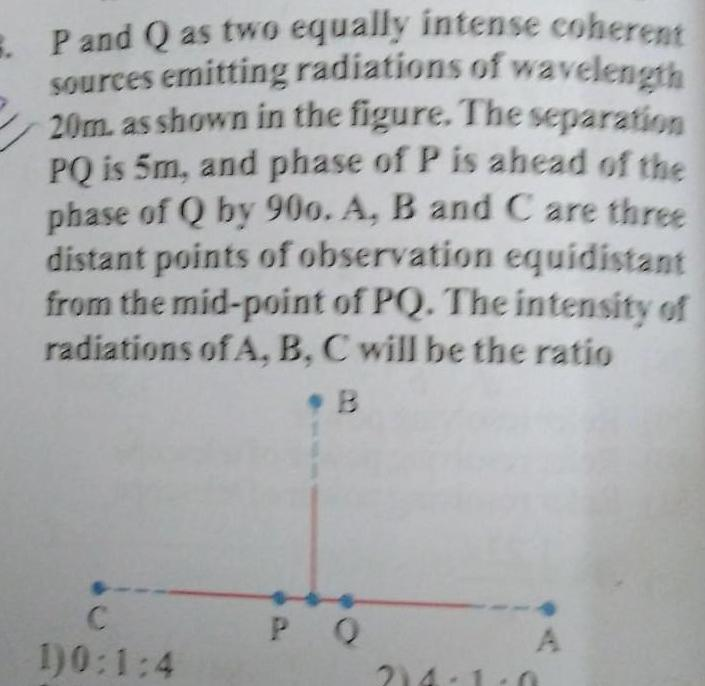Physics
Wave Optics
P and Q as two equally intense coherent sources emitting radiations of wavelength 20m as shown in the figure The separation PQ is 5m and phase of P is ahead of the phase of Q by 900 A B and C are three distant points of observation equidistant from the mid point of PQ The intensity of radiations of A B C will be the ratio B C 1 0 1 4 PQ A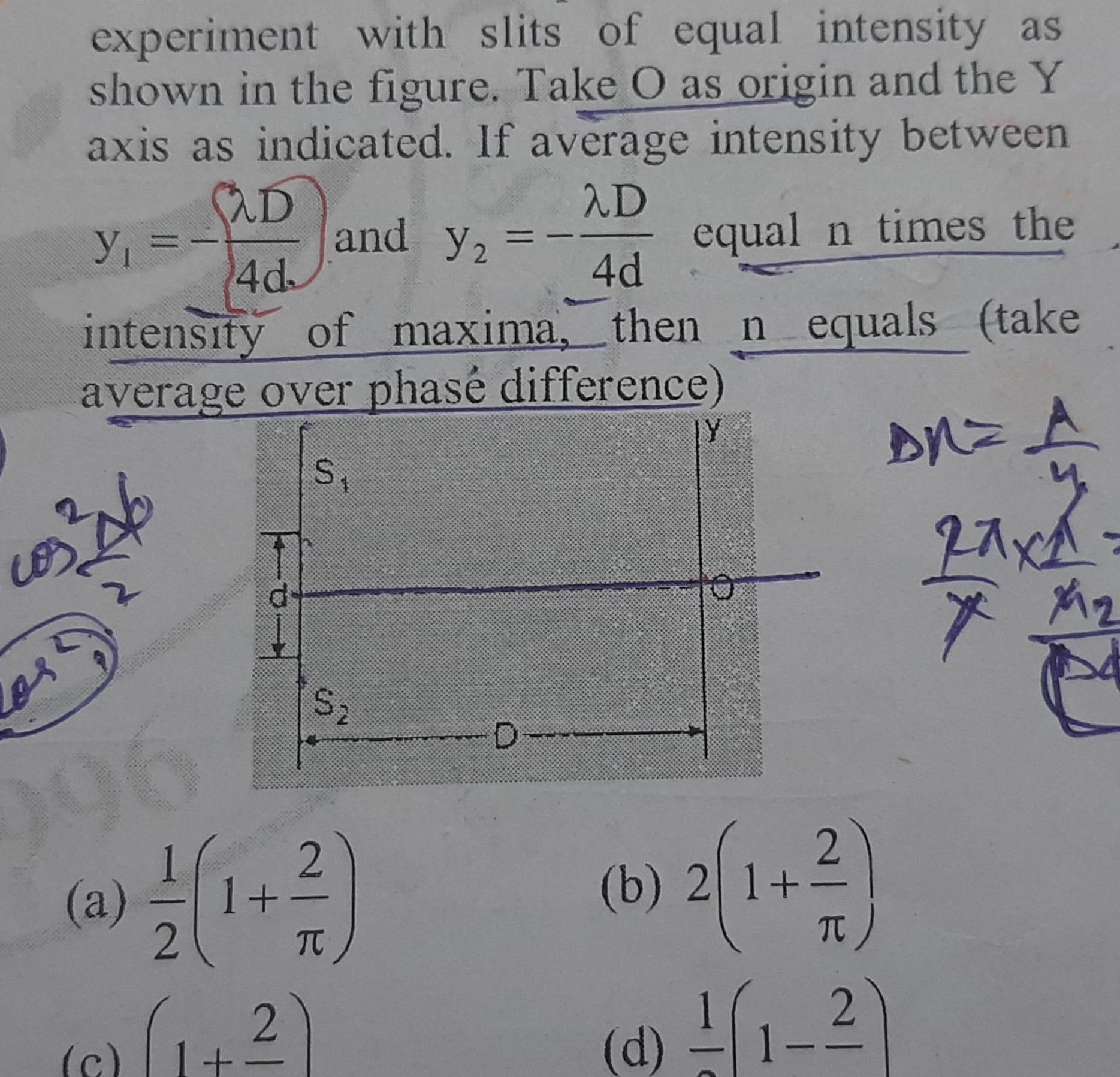Physics
Wave Optics
experiment with slits of equal intensity as shown in the figure Take O as origin and the Y axis as indicated If average intensity between D y and Y2 equal n times the 4d intensity of maxima then n equals take average over phas difference S os 21 90 1 a D 4d 2 d 1 S 2 T 1 2 D IY O AN A y 2axa X X 1 b 2 1 2 d 1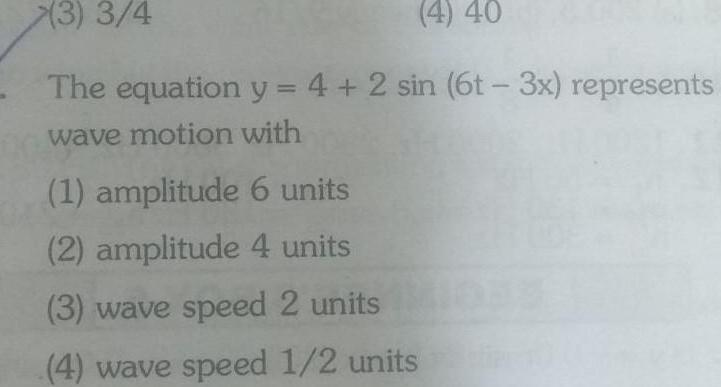Physics
Wave Optics
3 3 4 4 40 The equation y 4 2 sin 6t 3x represents wave motion with 1 amplitude 6 units 2 amplitude 4 units 3 wave speed 2 units 4 wave speed 1 2 units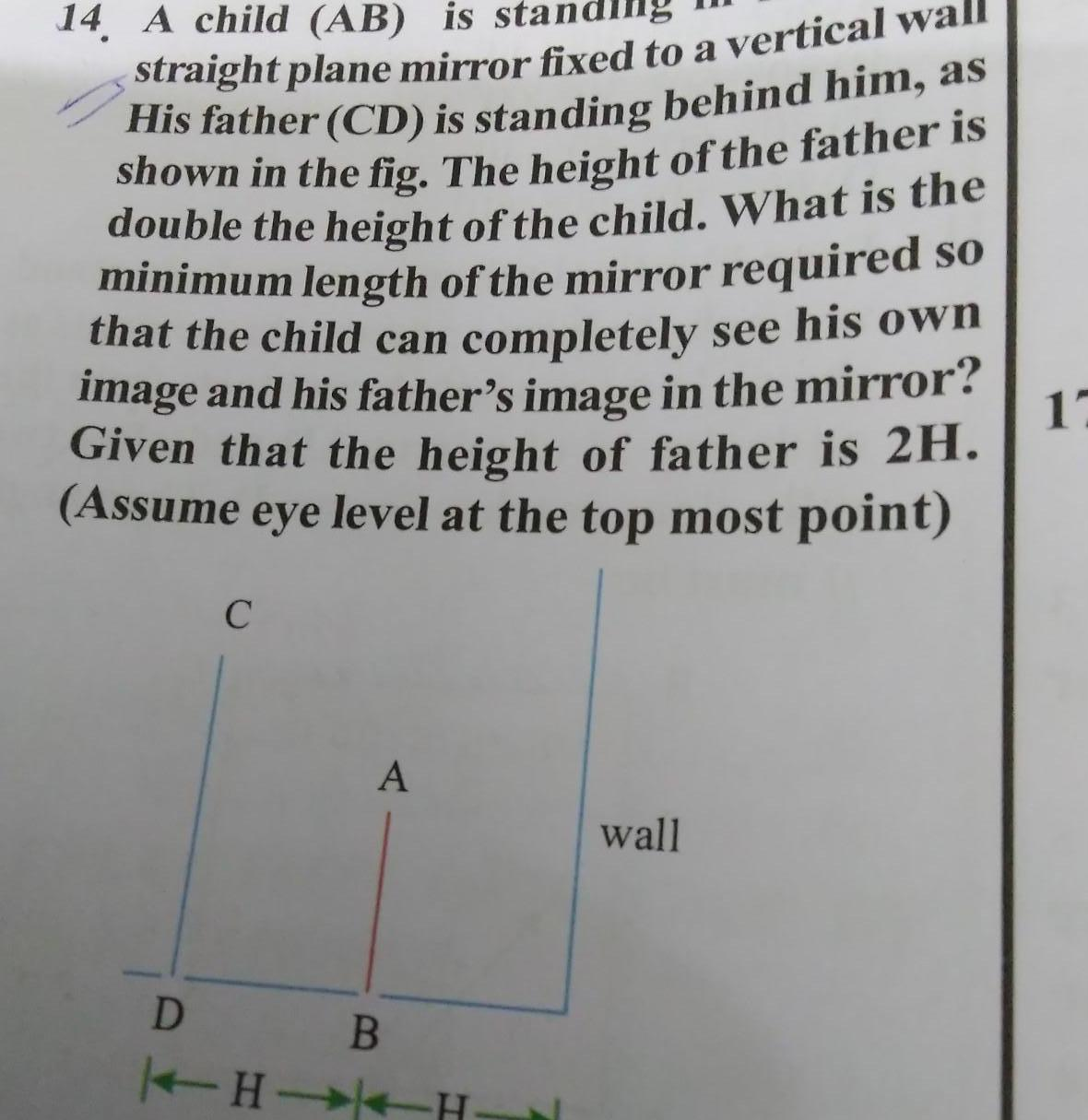Physics
Wave Optics
14 A child AB is star straight plane mirror fixed to a vertical wall His father CD is standing behind him as shown in the fig The height of the father is double the height of the child What is the minimum length of the mirror required so that the child can completely see his own image and his father s image in the mirror Given that the height of father is 2H Assume eye level at the top most point D C A B H H wall 12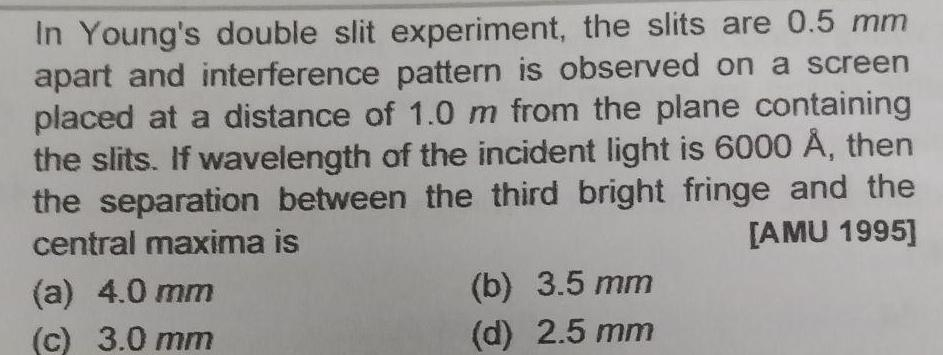Physics
Wave Optics
In Young s double slit experiment the slits are 0 5 mm apart and interference pattern is observed on a screen placed at a distance of 1 0 m from the plane containing the slits If wavelength of the incident light is 6000 then the separation between the third bright fringe and the central maxima is AMU 1995 a 4 0 mm c 3 0 mm b 3 5 mm d 2 5 mm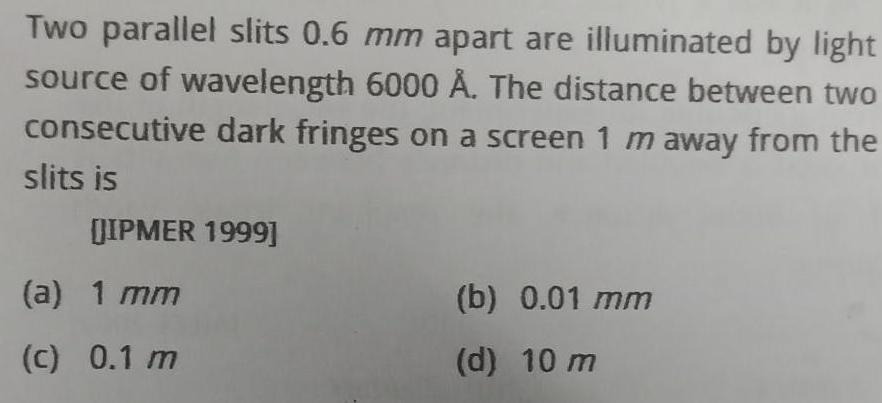Physics
Wave Optics
Two parallel slits 0 6 mm apart are illuminated by light source of wavelength 6000 The distance between two consecutive dark fringes on a screen 1 m away from the slits is JIPMER 1999 a 1 mm c 0 1 m b 0 01 mm d 10 m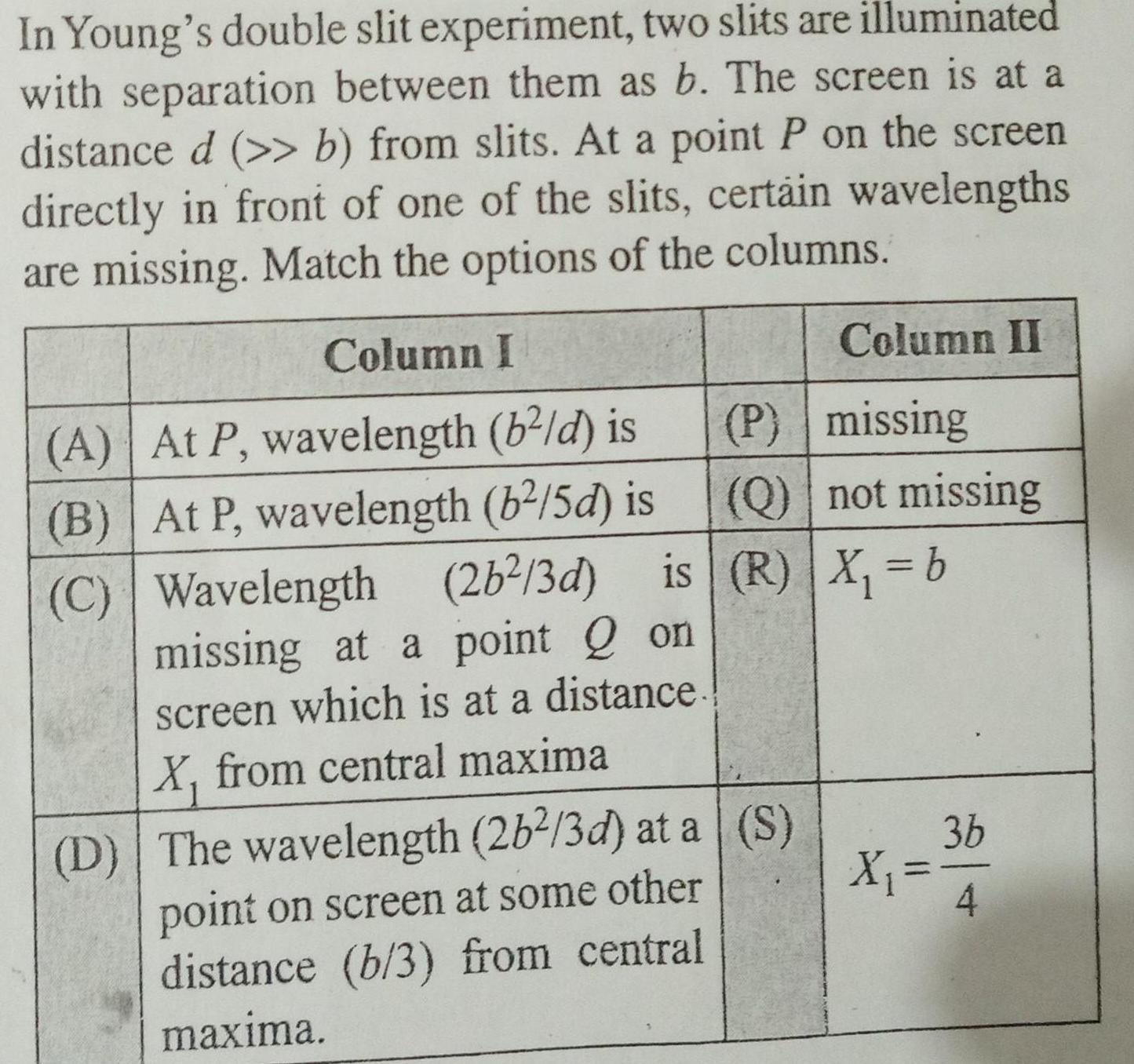Physics
Wave Optics
In Young s double slit experiment two slits are illuminated with separation between them as b The screen is at a distance d b from slits At a point P on the screen directly in front of one of the slits certain wavelengths are missing Match the options of the columns Column I A At P wavelength b d is B At P wavelength b 5d is C D The wavelength 26 3d at a point on screen at some other distance b 3 from central maxima Column II P missing Q not missing Wavelength 2b 3d is R X b missing at a point Q on screen which is at a distance X from central maxima S X 3b 4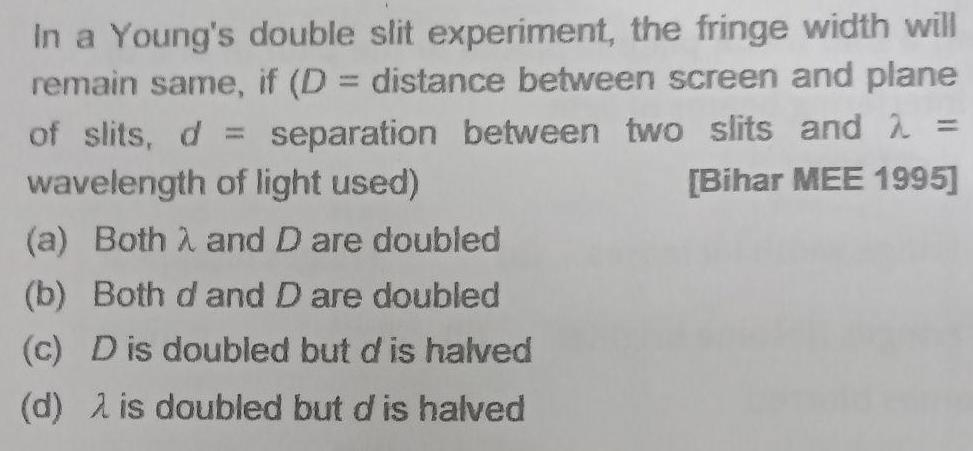Physics
Wave Optics
In a Young s double slit experiment the fringe width will remain same if D distance between screen and plane of slits d separation between two slits and wavelength of light used Bihar MEE 1995 a Both and D are doubled b Both d and D are doubled c Dis doubled but d is halved d 2 is doubled but d is halved 1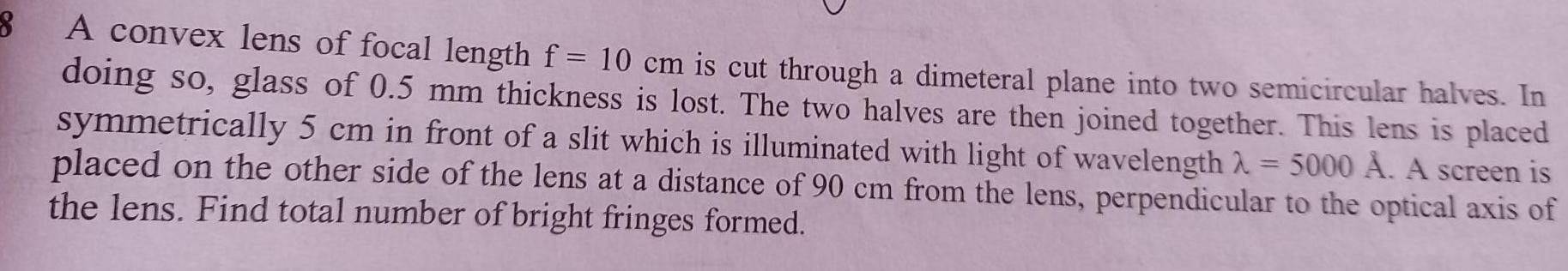Physics
Wave Optics
8 A convex lens of focal length f 10 cm is cut through a dimeteral plane into two semicircular halves In doing so glass of 0 5 mm thickness is lost The two halves are then joined together This lens is placed symmetrically 5 cm in front of a slit which is illuminated with light of wavelength 5000 A A screen is placed on the other side of the lens at a distance of 90 cm from the lens perpendicular to the optical axis of the lens Find total number of bright fringes formed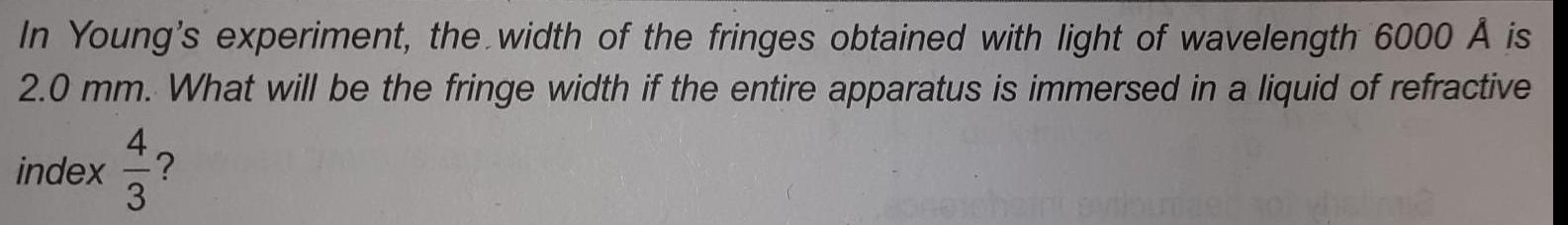Physics
Wave Optics
In Young s experiment the width of the fringes obtained with light of wavelength 6000 A is 2 0 mm What will be the fringe width if the entire apparatus is immersed in a liquid of refractive index 4 3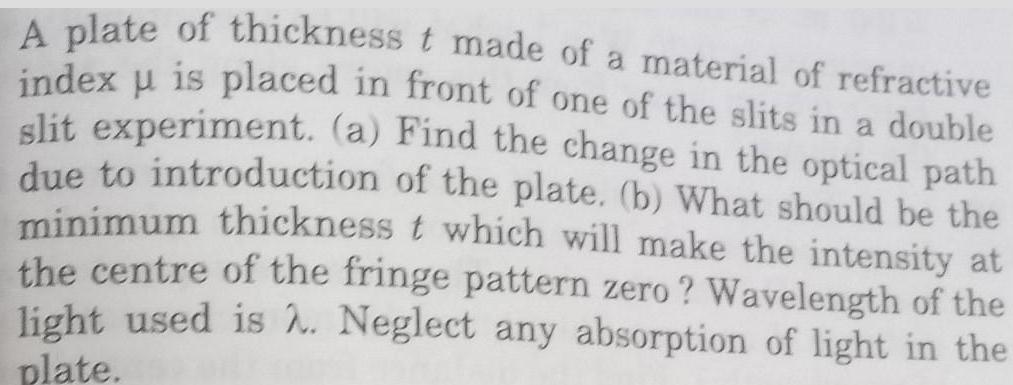Physics
Wave Optics
A plate of thickness t made of a material of refractive index u is placed in front of one of the slits in a double slit experiment a Find the change in the optical path due to introduction of the plate b What should be the minimum thickness t which will make the intensity at the centre of the fringe pattern zero Wavelength of the light used is 2 Neglect any absorption of light in the plate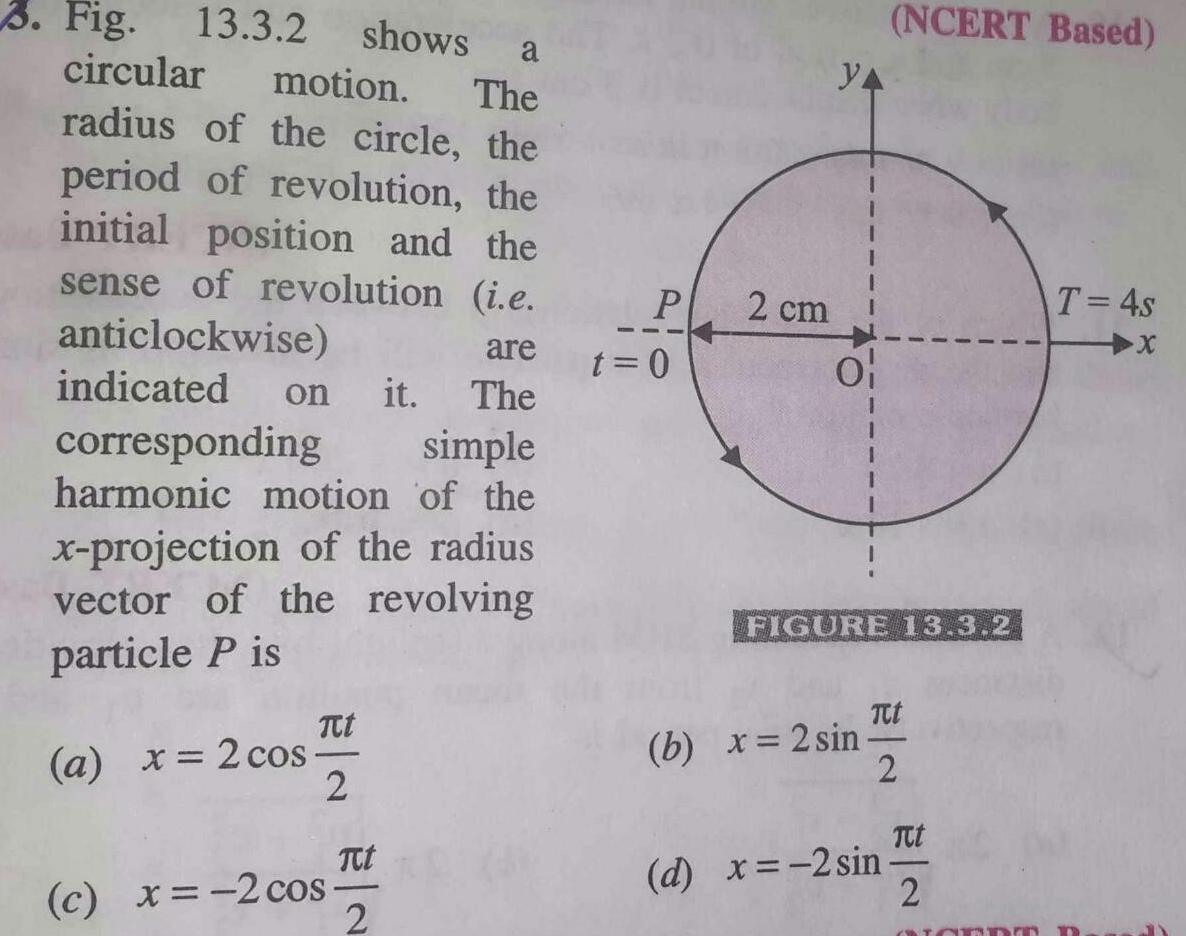Physics
Wave Optics
3 Fig 13 3 2 shows a circular motion The radius of the circle the period of revolution the initial position and the sense of revolution i e anticlockwise are The indicated on it corresponding simple harmonic motion of the x projection of the radius vector of the revolving particle P is Tt a x 2 cos 2 c x 2 cos TCt 2 P t 0 2 cm YA FIGURE 13 3 2 b x 2 sin NCERT Based Ttt 2 d x 2 sin Tut 2 T 4s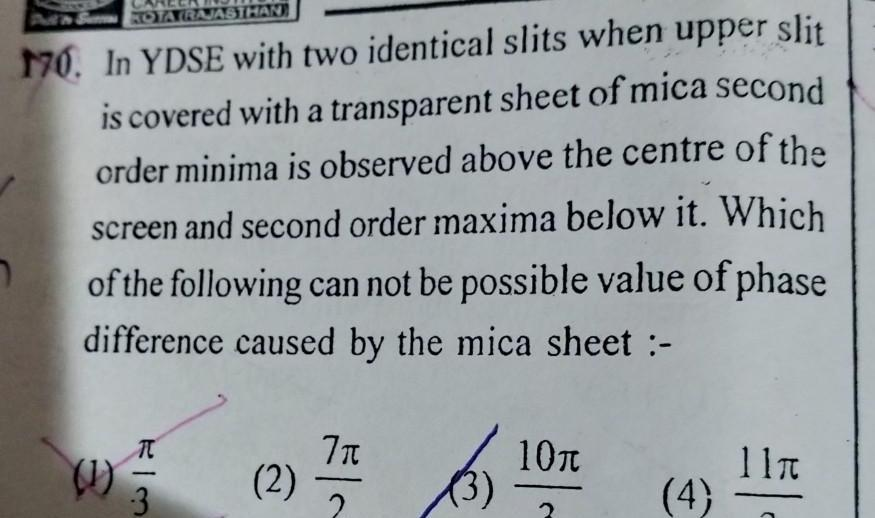Physics
Wave Optics
KOTA RAJASTHAN 170 In YDSE with two identical slits when upper slit is covered with a transparent sheet of mica second order minima is observed above the centre of the screen and second order maxima below it Which of the following can not be possible value of phase difference caused by the mica sheet 1 FIM 3 2 7 3 10 2 4 11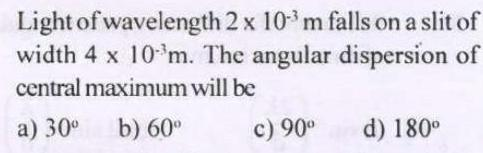Physics
Wave Optics
Light of wavelength 2 x 10 m falls on a slit of width 4 x 10 m The angular dispersion of central maximum will be a 30 b 60 c 90 d 180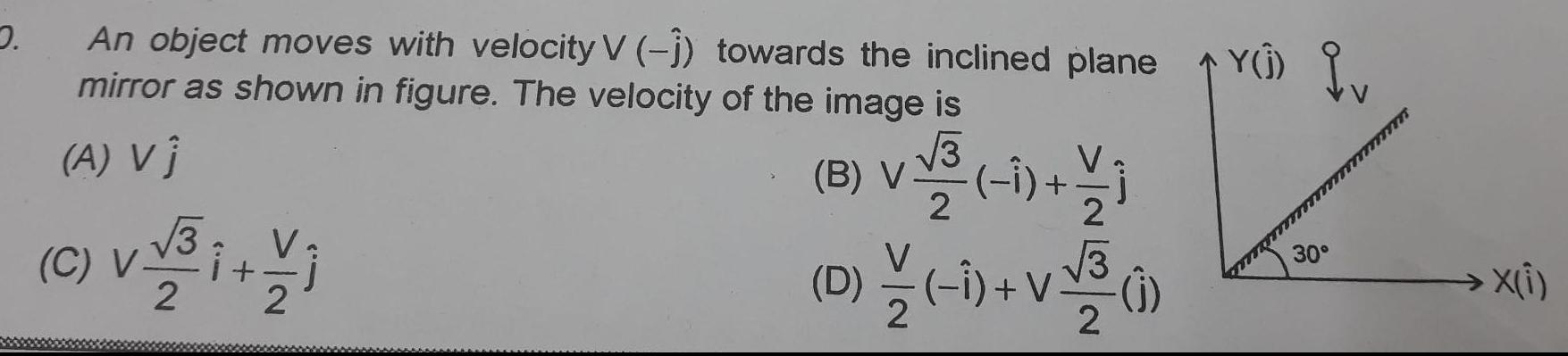Physics
Wave Optics
2 An object moves with velocity V 1 towards the inclined plane mirror as shown in figure The velocity of the image is A Vi B V C 3i 2 2 1 D 1 V 3 Y Iv 30 x i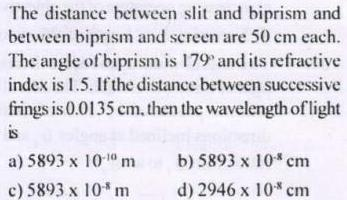Physics
Wave Optics
The distance between slit and biprism and between biprism and screen are 50 cm each The angle of biprism is 179 and its refractive index is 1 5 If the distance between successive frings is 0 0135 cm then the wavelength of light is a 5893 x 10 0 m c 5893 x 10 m b 5893 x 10 cm d 2946 x 10 cm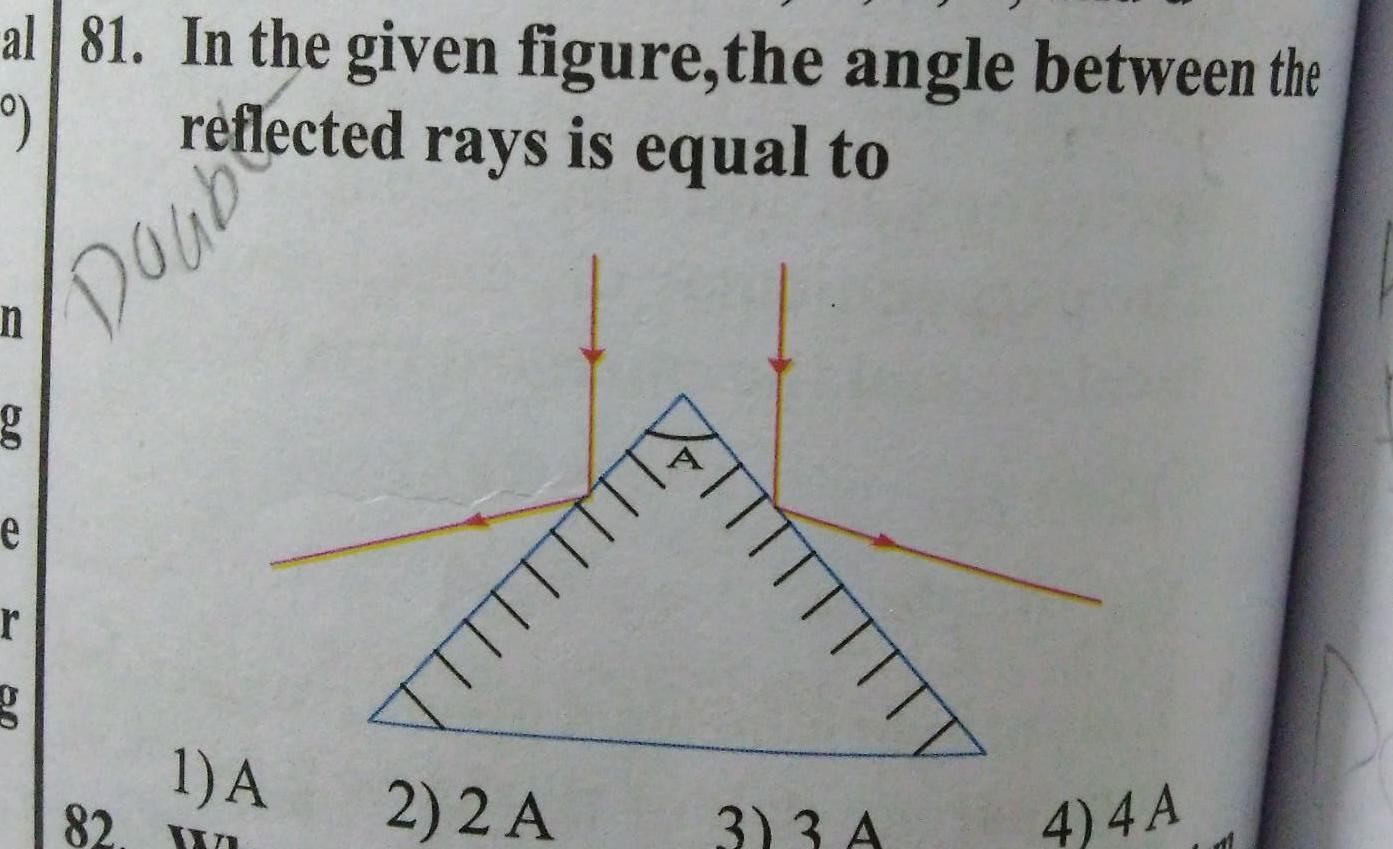Physics
Wave Optics
al 81 In the given figure the angle between the 9 Dalected rays is equal to n g e r 82 1 A 2 2 A 3 3 A 4 4A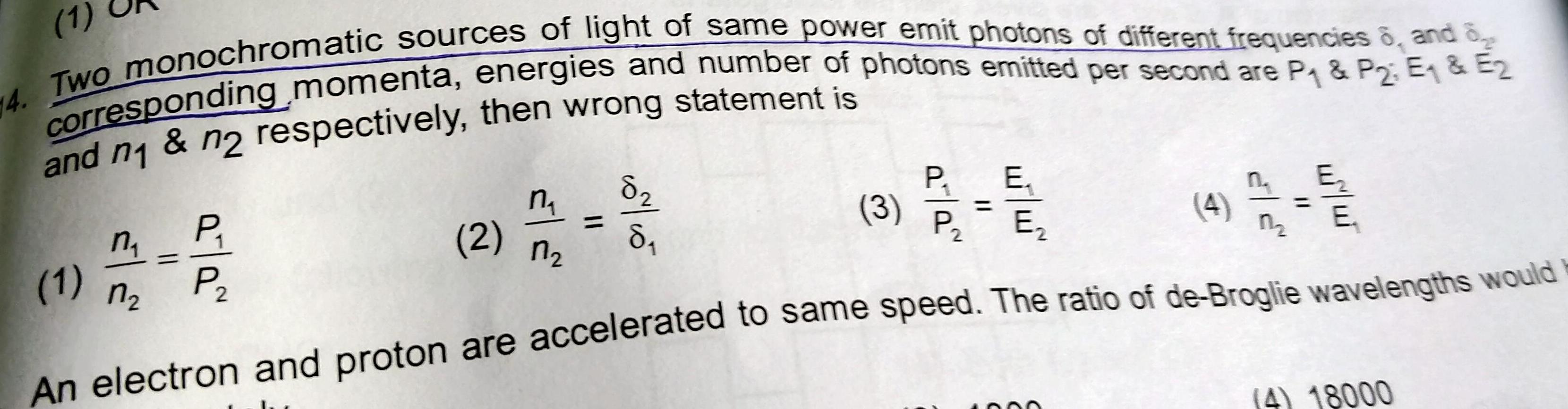Physics
Wave Optics
1 14 Two monochromatic sources of light of same power emit photons of different frequencies 8 and 8 corresponding momenta energies and number of photons emitted per second are P P2 E E and n1 n respectively then wrong statement is P E 3 P E 2 An electron and proton are accelerated to same speed The ratio of de Broglie wavelengths would M P a a 1 n 2 n 10 10 1 8 E n 4 18000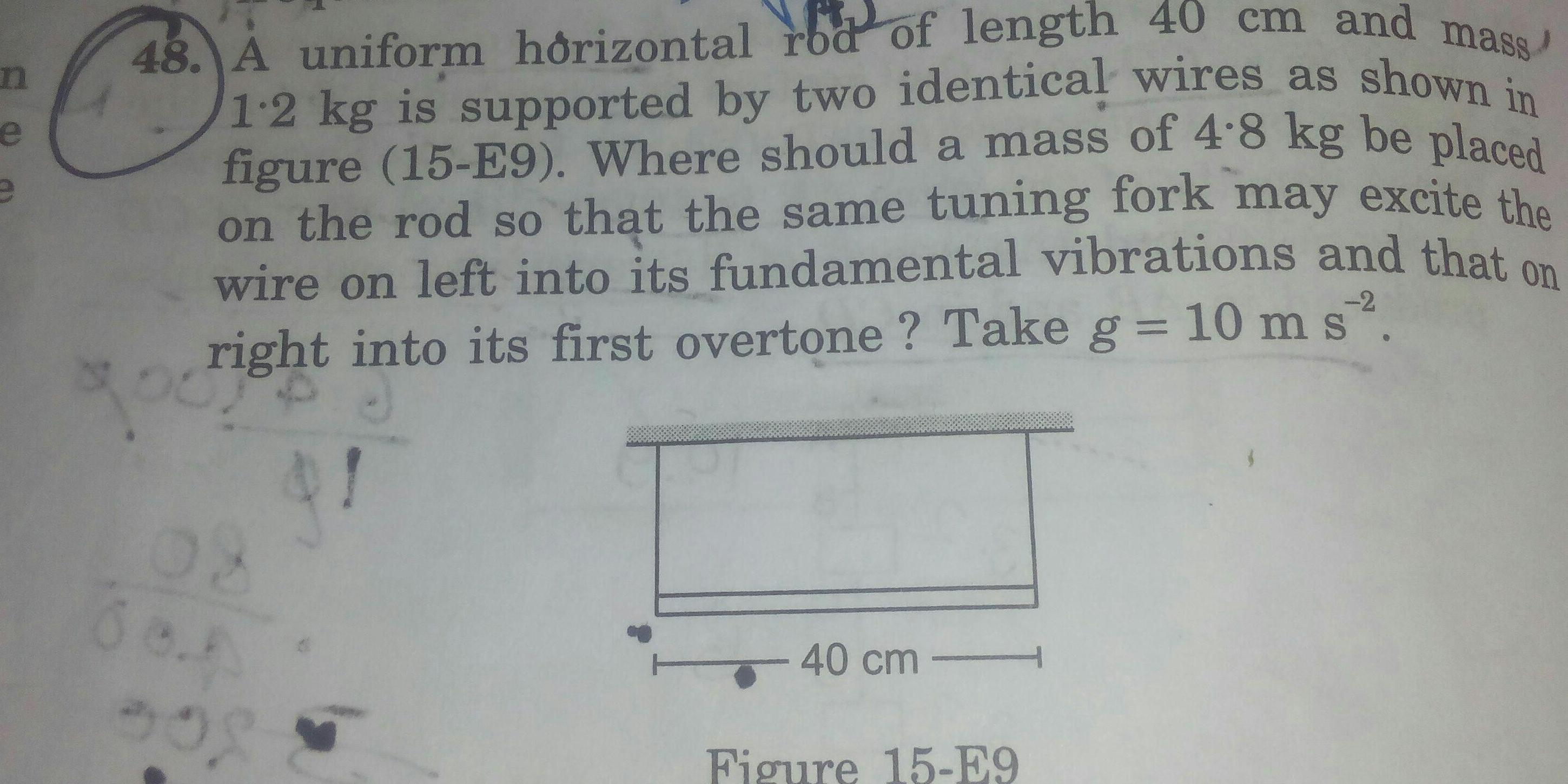Physics
Wave Optics
n e C 48 A uniform horizontal rod of length 40 cm and mass 1 2 kg is supported by two identical wires as shown in figure 15 E9 Where should a mass of 4 8 kg be placed on the rod so that the same tuning fork may excite the wire on left into its fundamental vibrations and that on right into its first overtone Take g 10 m s2 2 800 308 40 cm Figure 15 E9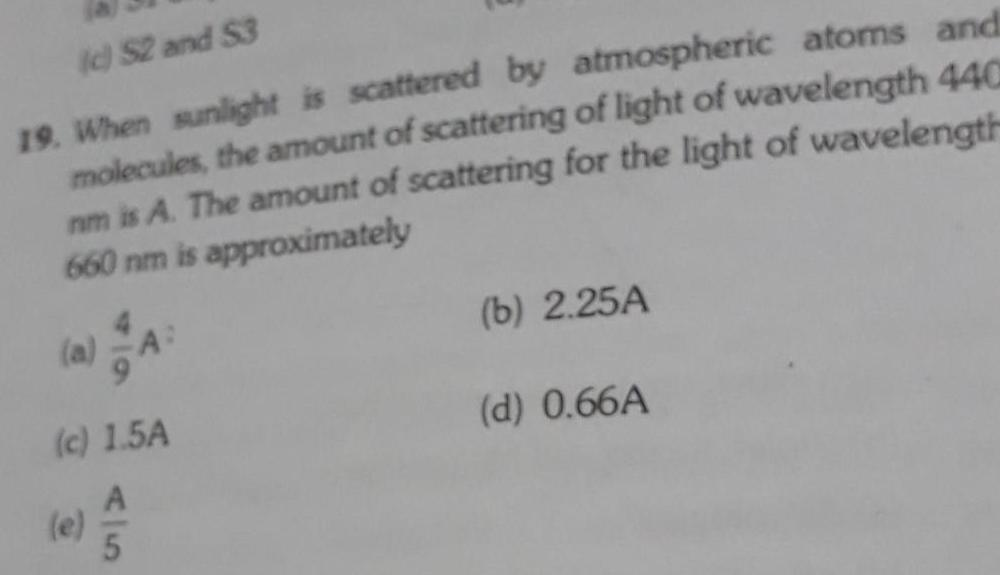Physics
Wave Optics
c S2 and 53 19 When sunlight is scattered by atmospheric atoms and molecules the amount of scattering of light of wavelength 440 nm is A The amount of scattering for the light of wavelength 660 nm is approximately c 1 5A A b 2 25A d 0 66A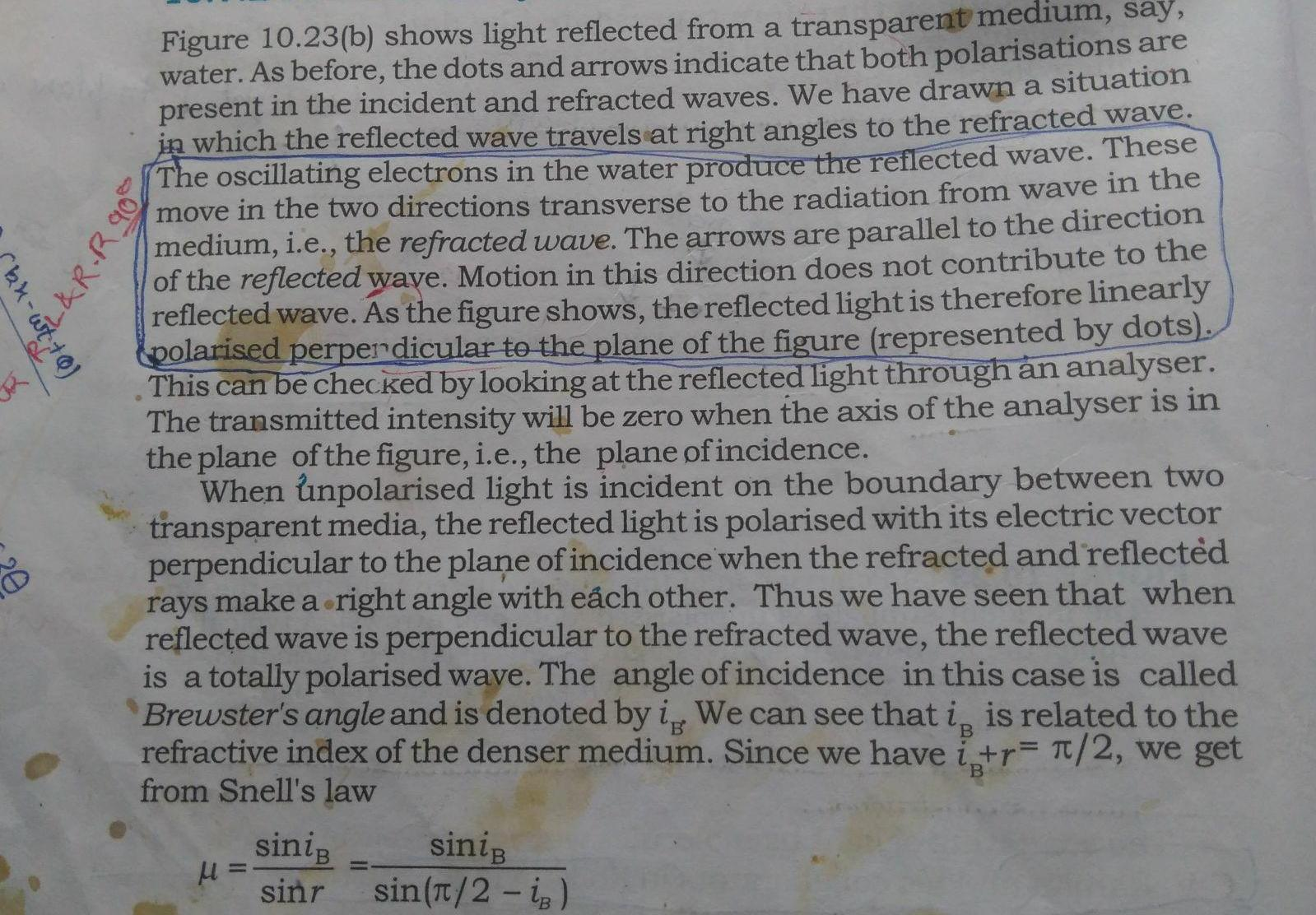Physics
Wave Optics
kk w wt Q Figure 10 23 b shows light reflected from a transparent medium say water As before the dots and arrows indicate that both polarisations are present in the incident and refracted waves We have drawn a situation in which the reflected wave travels at right angles to the refracted wave The oscillating electrons in the water produce the reflected wave These move in the two directions transverse to the radiation from wave in the medium i e the refracted wave The arrows are parallel to the direction of the reflected wave Motion in this direction does not contribute to the reflected wave As the figure shows the reflected light is therefore linearly polarised perpendicular to the plane of the figure represented by dots This can be checked by looking at the reflected light through an analyser The transmitted intensity will be zero when the axis of the analyser is in the plane of the figure i e the plane of incidence When unpolarised light is incident on the boundary between two transparent media the reflected light is polarised with its electric vector perpendicular to the plane of incidence when the refracted and reflected rays make a right angle with each other Thus we have seen that when reflected wave is perpendicular to the refracted wave the reflected wave is a totally polarised wave The angle of incidence in this case is called Brewster s angle and is denoted by ig We can see that i is related to the refractive index of the denser medium Since we have i r 2 we get from Snell s law R R 90 siniB sinr siniB sin 2 iB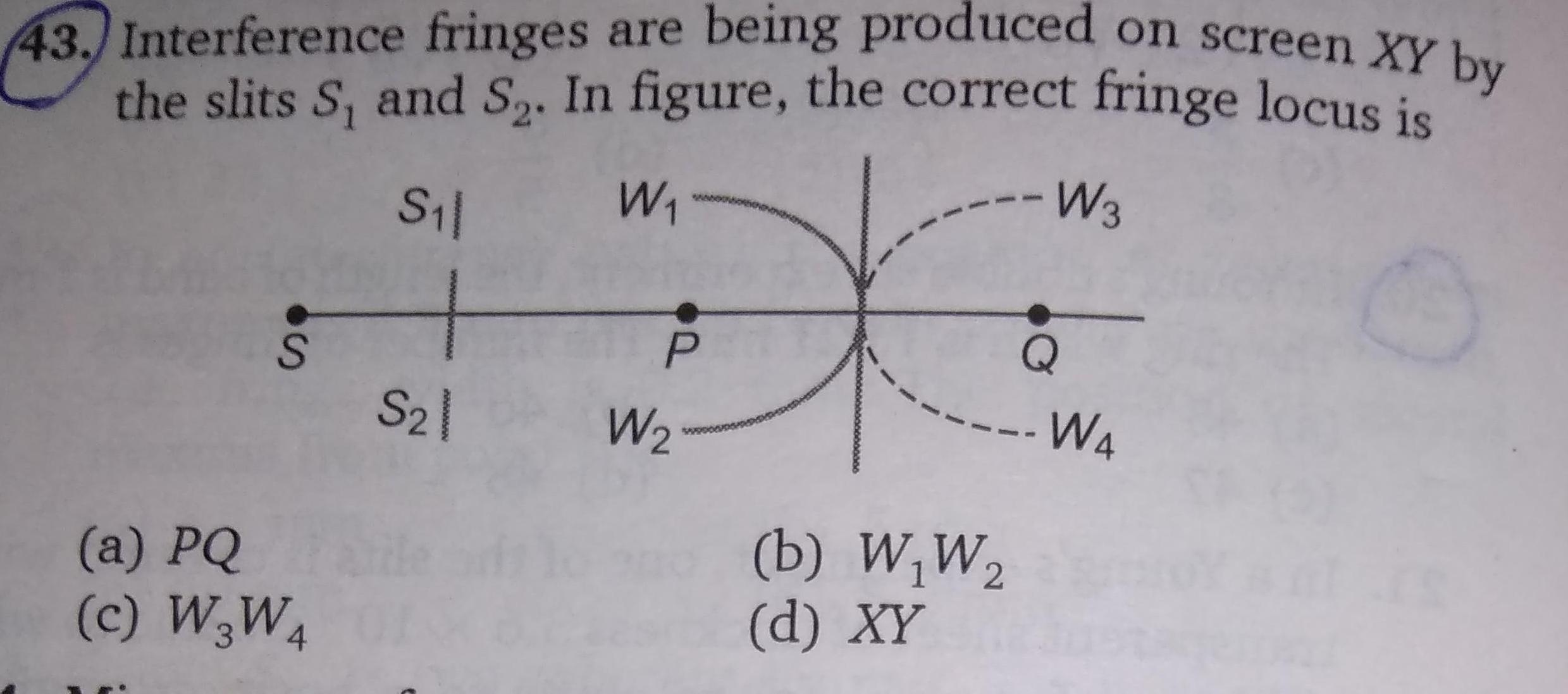Physics
Wave Optics
43 Interference fringes are being produced on screen XY by the slits S and S In figure the correct fringe locus is S W3 S a PQ C W3W4 S 1 2 W P W b W W d XY Q W4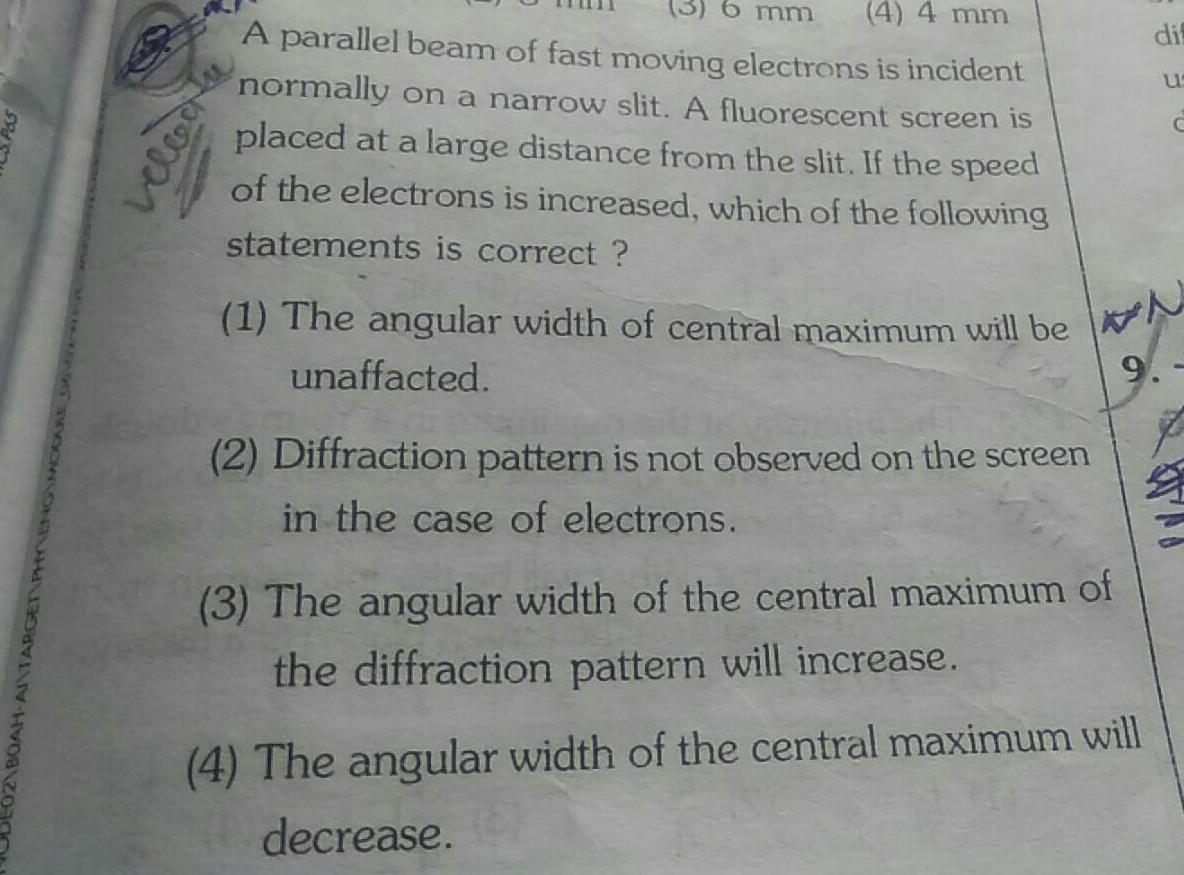Physics
Wave Optics
DE021B0AH ANTARGE 3 6 mm 4 4 mm A parallel beam of fast moving electrons is incident normally on a narrow slit A fluorescent screen is placed at a large distance from the slit If the speed of the electrons is increased which of the following statements is correct 1 The angular width of central maximum will be N unaffacted 9 2 Diffraction pattern is not observed on the screen in the case of electrons cleach 3 The angular width of the central maximum of the diffraction pattern will increase 4 The angular width of the central maximum will decrease dif us C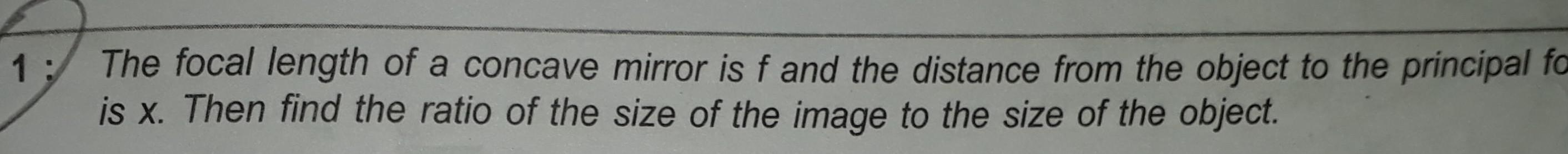Physics
Wave Optics
1 The focal length of a concave mirror is f and the distance from the object to the principal fo is x Then find the ratio of the size of the image to the size of the object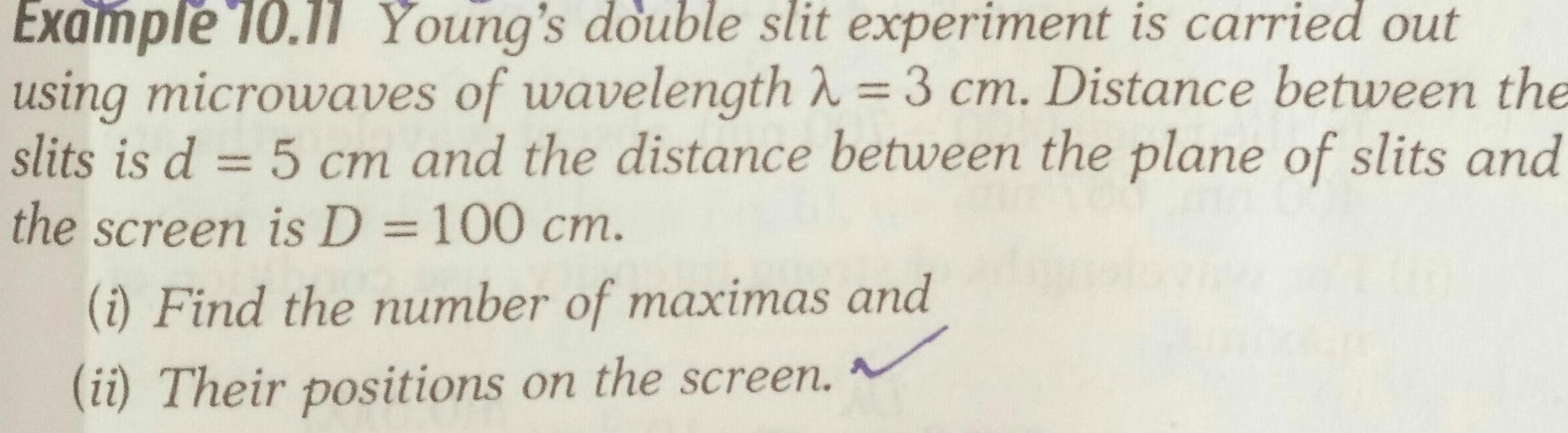Physics
Wave Optics
Example 10 11 Young s double slit experiment is carried out using microwaves of wavelength 3 cm Distance between the slits is d 5 cm and the distance between the plane of slits and the screen is D 100 cm i Find the number of maximas and ii Their positions on the screen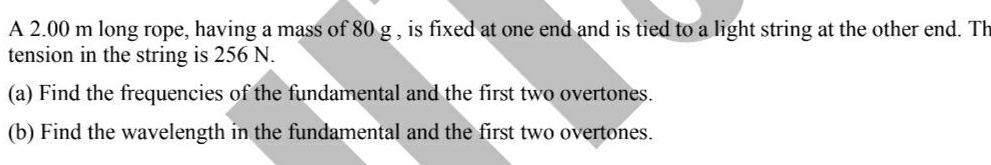Physics
Wave Optics
A 2 00 m long rope having a mass of 80 g is fixed at one end and is tied to a light string at the other end Th tension in the string is 256 N a Find the frequencies of the fundamental and the first two overtones b Find the wavelength in the fundamental and the first two overtones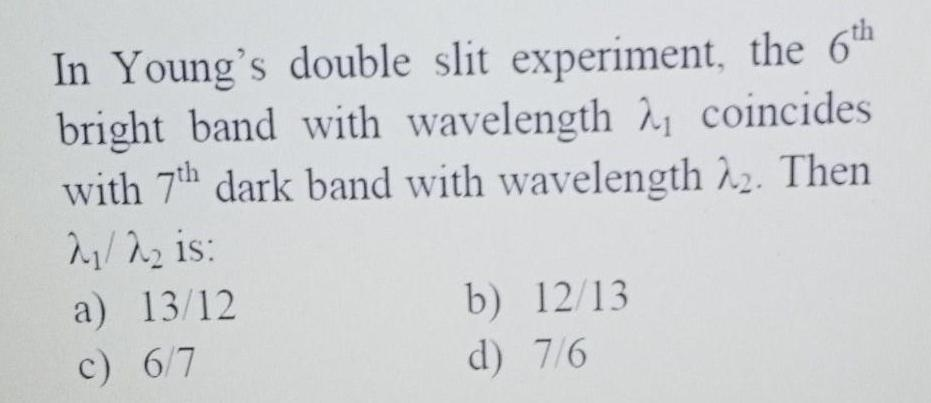Physics
Wave Optics
In Young s double slit experiment the 6th bright band with wavelength coincides with 7th dark band with wavelength 22 Then 2 is a 13 12 c 6 7 b 12 13 d 7 6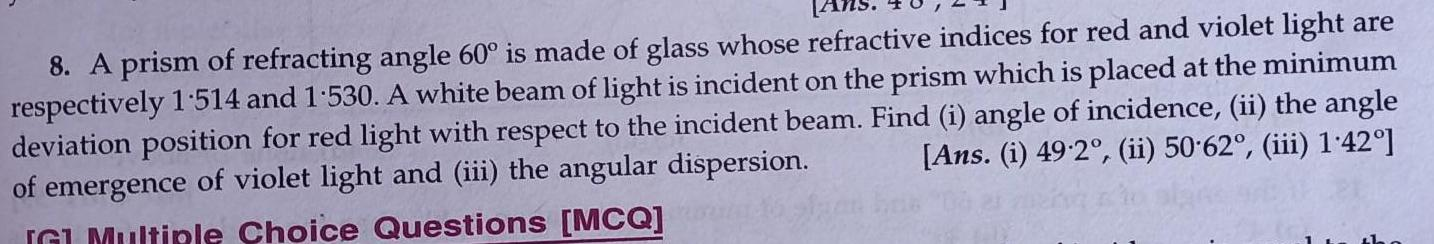Physics
Wave Optics
8 A prism of refracting angle 60 is made of glass whose refractive indices for red and violet light are respectively 1 514 and 1 530 A white beam of light is incident on the prism which is placed at the minimum deviation position for red light with respect to the incident beam Find i angle of incidence ii the angle Ans i 49 2 ii 50 62 iii 1 42 of emergence of violet light and iii the angular dispersion G Multiple Choice Questions MCQ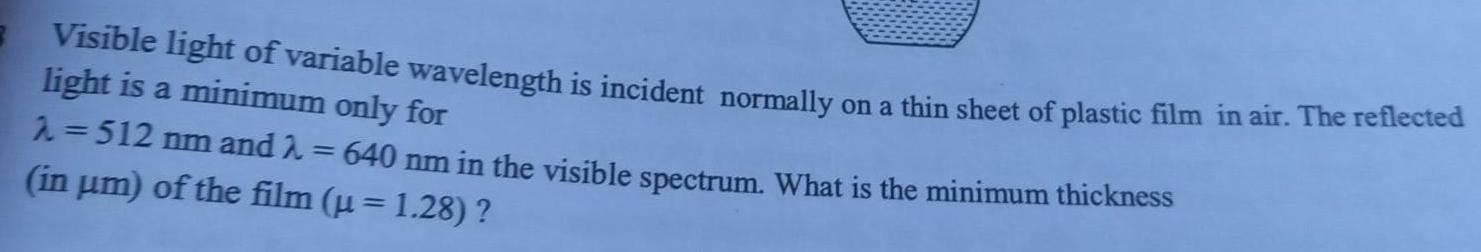Physics
Wave Optics
Visible light of variable wavelength is incident normally on a thin sheet of plastic film in air The reflected light is a minimum only for 512 nm and in um of the film u 1 28 640 nm in the visible spectrum What is the minimum thickness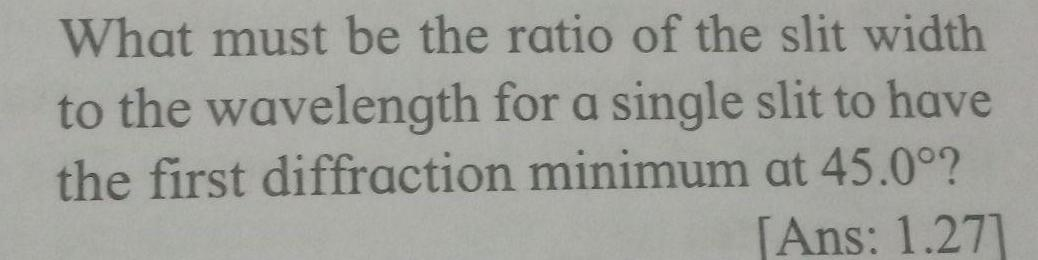Physics
Wave Optics
What must be the ratio of the slit width to the wavelength for a single slit to have the first diffraction minimum at 45 0 Ans 1 27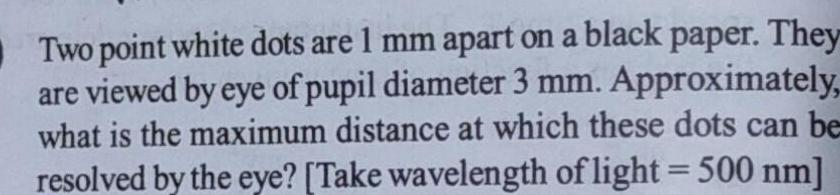Physics
Wave Optics
Two point white dots are 1 mm apart on a black paper They are viewed by eye of pupil diameter 3 mm Approximately what is the maximum distance at which these dots can be resolved by the eye Take wavelength of light 500 nm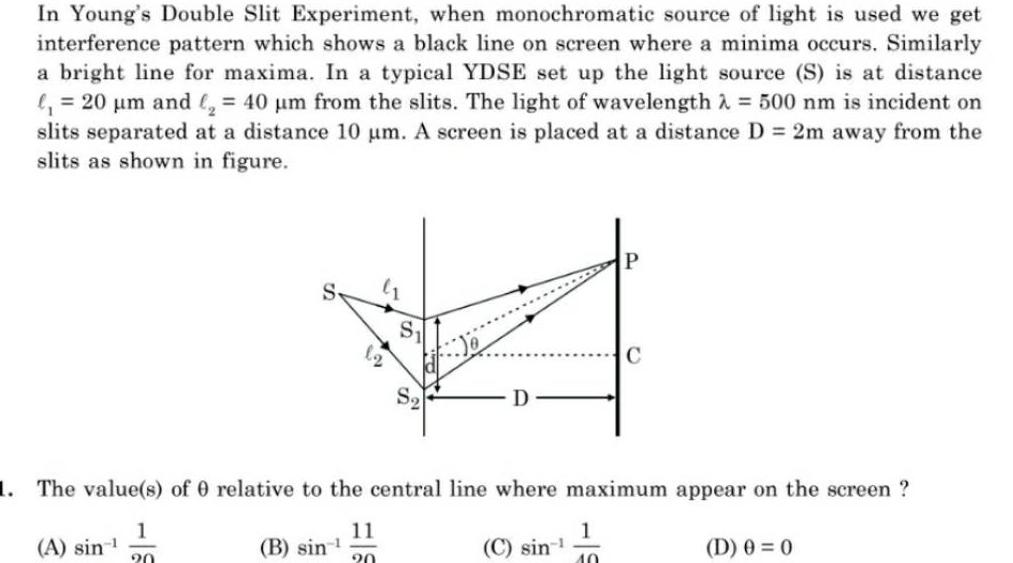Physics
Wave Optics
In Young s Double Slit Experiment when monochromatic source of light is used we get interference pattern which shows a black line on screen where a minima occurs Similarly a bright line for maxima In a typical YDSE set up the light source S is at distance 20 m and 40 m from the slits The light of wavelength 500 nm is incident on slits separated at a distance 10 m A screen is placed at a distance D 2m away from the slits as shown in figure S A sin D B sin 1 The value s of 0 relative to the central line where maximum appear on the screen 1 11 D 0 0 20 20 C sin P 1 10 C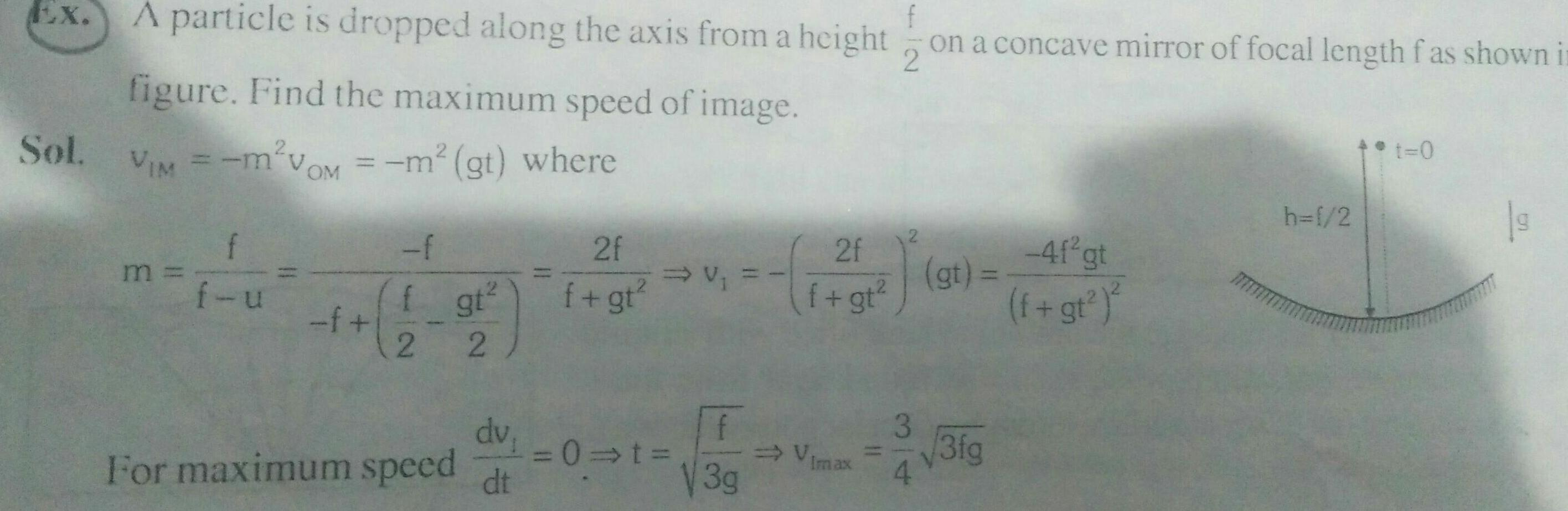Physics
Wave Optics
EX A particle is dropped along the axis from a height f figure Find the maximum speed of image Sol VIM m VOM m gt where f f u f f f gt 2 2 dv For maximum speed dt 11 2f f gt v 0 t 3g 2f f gt V Imax on a concave mirror of focal length fas shown in 2 3 4 gt 3fg 4f gt f gt h 1 2 t 0 19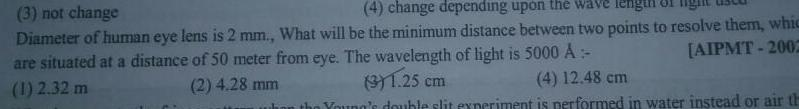Physics
Wave Optics
4 change depending upon the wave length 3 not change Diameter of human eye lens is 2 mm What will be the minimum distance between two points to resolve them which are situated at a distance of 50 meter from eye The wavelength of light is 5000 A 1 2 32 m AIPMT 2002 3 1 25 cm 4 12 48 cm 2 4 28 mm when the Young s double slit experiment is performed in water instead or air th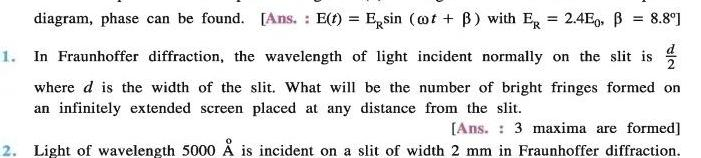Physics
Wave Optics
diagram phase can be found Ans E t Esin t B with ER 2 4E0 B 8 8 1 In Fraunhoffer diffraction the wavelength of light incident normally on the slit is where d is the width of the slit What will be the number of bright fringes formed on an infinitely extended screen placed at any distance from the slit Ans 3 maxima are formed 2 Light of wavelength 5000 A is incident on a slit of width 2 mm in Fraunhoffer diffraction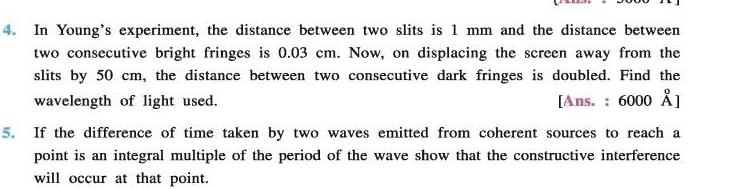Physics
Wave Optics
4 In Young s experiment the distance between two slits is 1 mm and the distance between two consecutive bright fringes is 0 03 cm Now on displacing the screen away from the slits by 50 cm the distance between two consecutive dark fringes is doubled Find the wavelength of light used Ans 6000 5 If the difference of time taken by two waves emitted from coherent sources to reach a point is an integral multiple of the period of the wave show that the constructive interference will occur at that point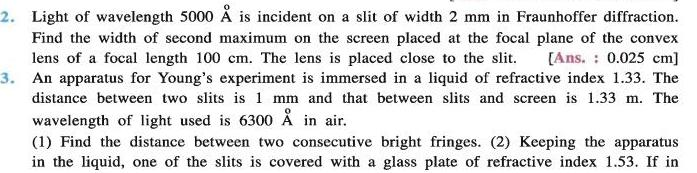Physics
Wave Optics
2 Light of wavelength 5000 is incident on a slit of width 2 mm in Fraunhoffer diffraction Find the width of second maximum on the screen placed at the focal plane of the convex lens of a focal length 100 cm The lens is placed close to the slit Ans 0 025 cm 3 An apparatus for Young s experiment is immersed in a liquid of refractive index 1 33 The distance between two slits is 1 mm and that between slits and screen is 1 33 m The wavelength of light used is 6300 in air 1 Find the distance between two consecutive bright fringes 2 Keeping the apparatus in the liquid one of the slits is covered with a glass plate of refractive index 1 53 If in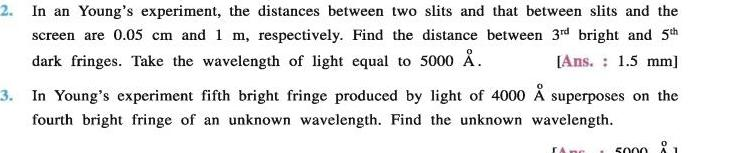Physics
Wave Optics
2 In an Young s experiment the distances between two slits and that between slits and the screen are 0 05 cm and 1 m respectively Find the distance between 3rd bright and 5th dark fringes Take the wavelength of light equal to 5000 Ans 1 5 mm 3 In Young s experiment fifth bright fringe produced by light of 4000 superposes on the fourth bright fringe of an unknown wavelength Find the unknown wavelength Anc 5000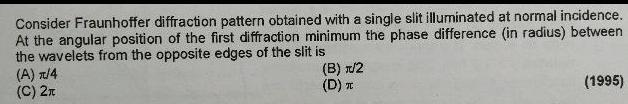Physics
Wave Optics
Consider Fraunhoffer diffraction pattern obtained with a single slit illuminated at normal incidence At the angular position of the first diffraction minimum the phase difference in radius between the wavelets from the opposite edges of the slit is A 4 C 2 B 1 2 D T 1995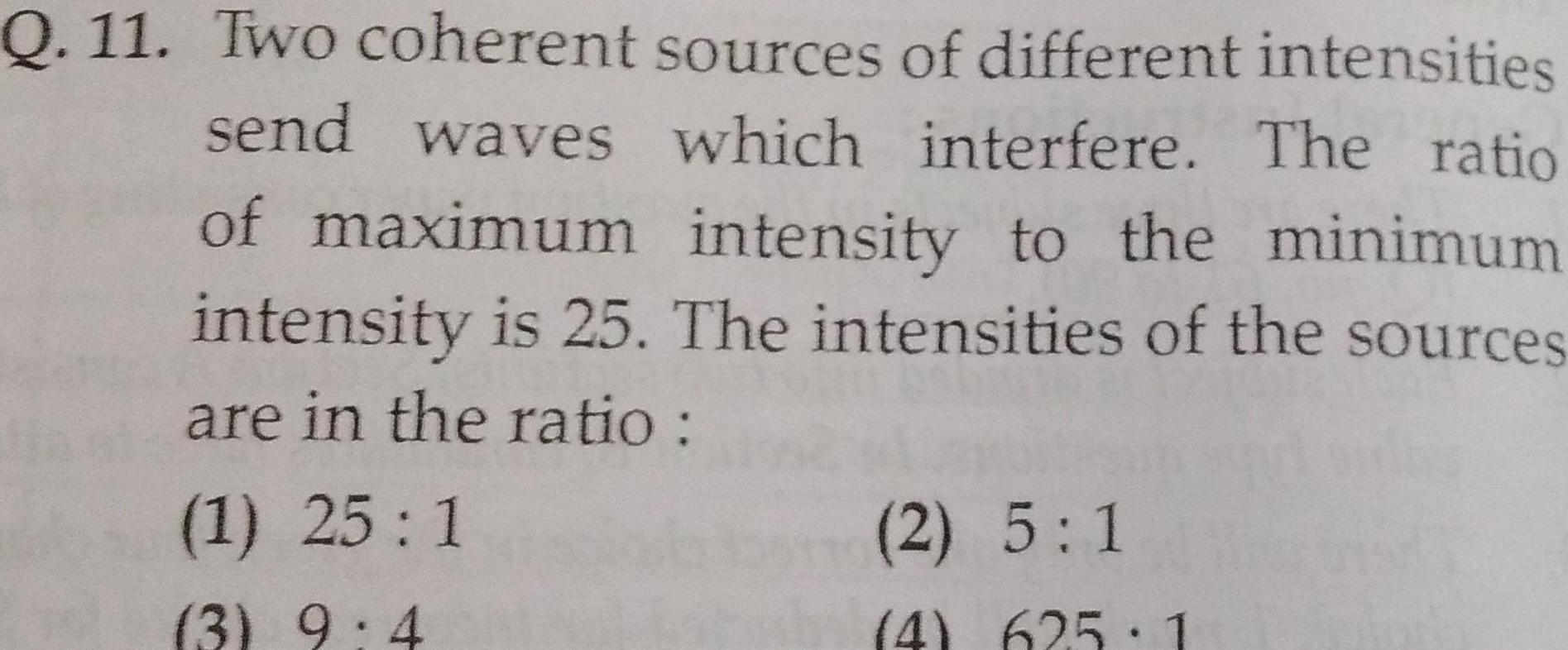Physics
Wave Optics
Q 11 Two coherent send waves sources of different intensities which interfere The ratio of maximum intensity to the minimum intensity is 25 The intensities of the sources are in the ratio 1 25 1 3 9 4 2 5 1 4 625 1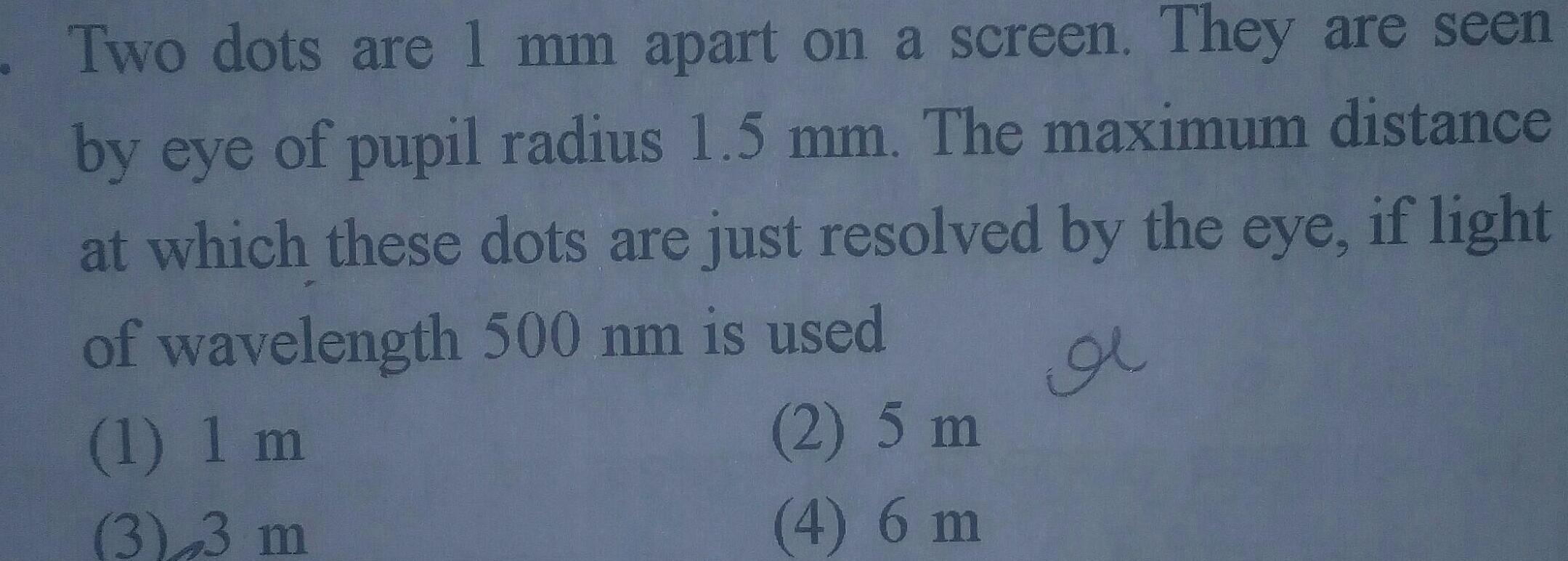Physics
Wave Optics
Two dots are 1 mm apart on a screen They are seen by eye of pupil radius 1 5 mm The maximum distance at which these dots are just resolved by the eye if light of wavelength 500 nm is used 1 1 m 2 5 m 4 6 m 3 3 m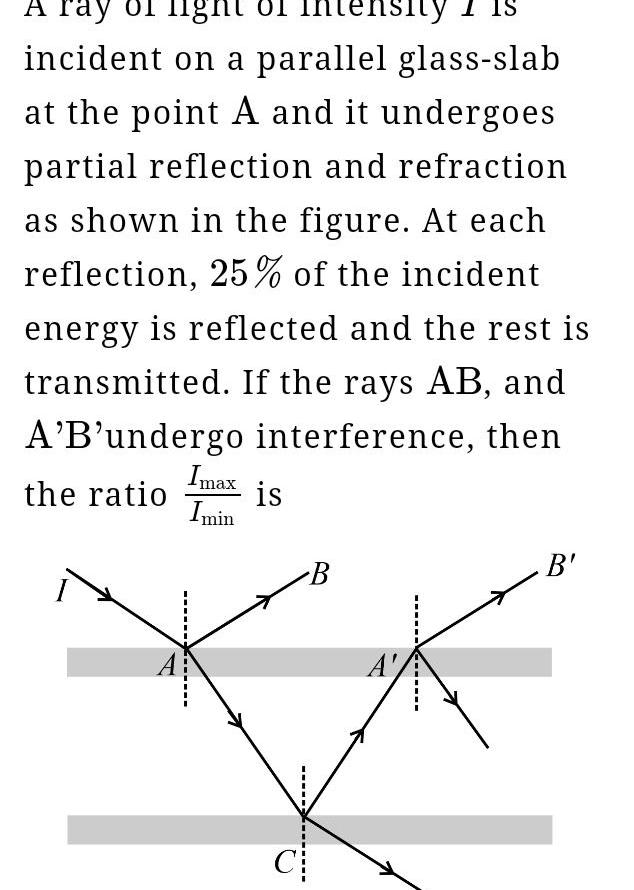Physics
Wave Optics
A ray light of intensity incident on a parallel glass slab at the point A and it undergoes partial reflection and refraction as shown in the figure At each reflection 25 of the incident energy is reflected and the rest is transmitted If the rays AB and A B undergo interference then the ratio Imax Imin is C B A B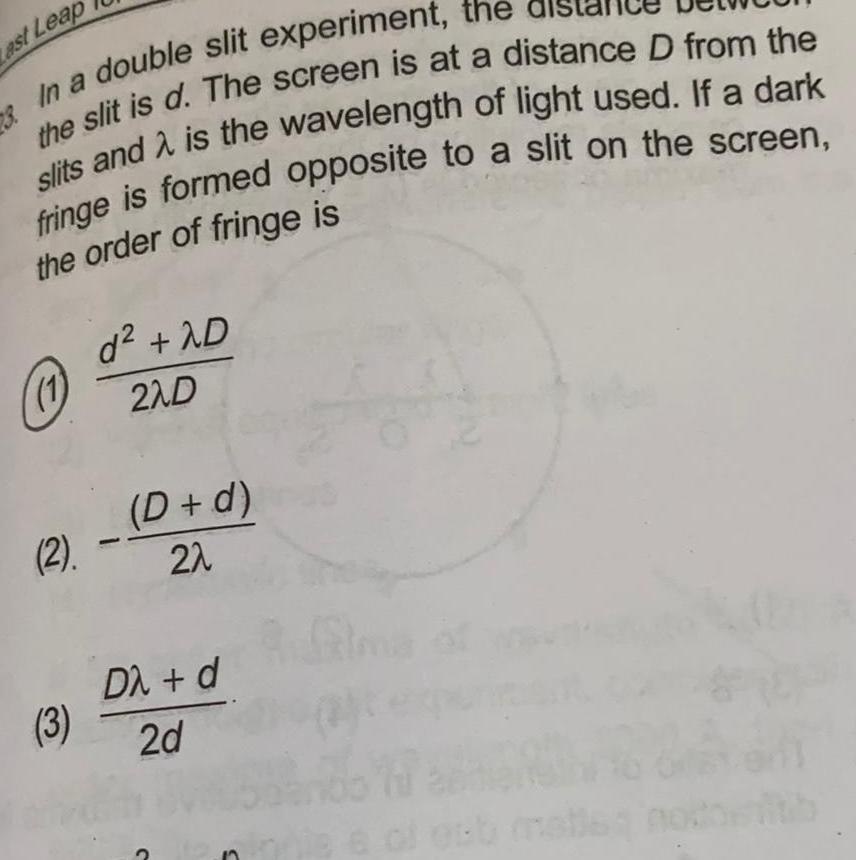Physics
Wave Optics
Last Leap 3 In a double slit experiment the the slit is d The screen is at a distance D from the slits and is the wavelength of light used If a dark fringe is formed opposite to a slit on the screen the order of fringe is 2 3 d 2D 2ND D d 2 D d 2d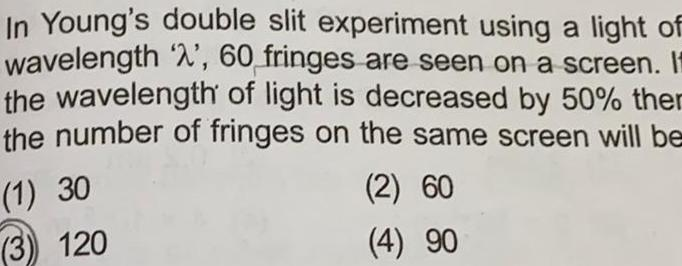Physics
Wave Optics
In Young s double slit experiment using a light of wavelength 2 60 fringes are seen on a screen It the wavelength of light is decreased by 50 ther the number of fringes on the same screen will be 1 30 2 60 4 90 3 120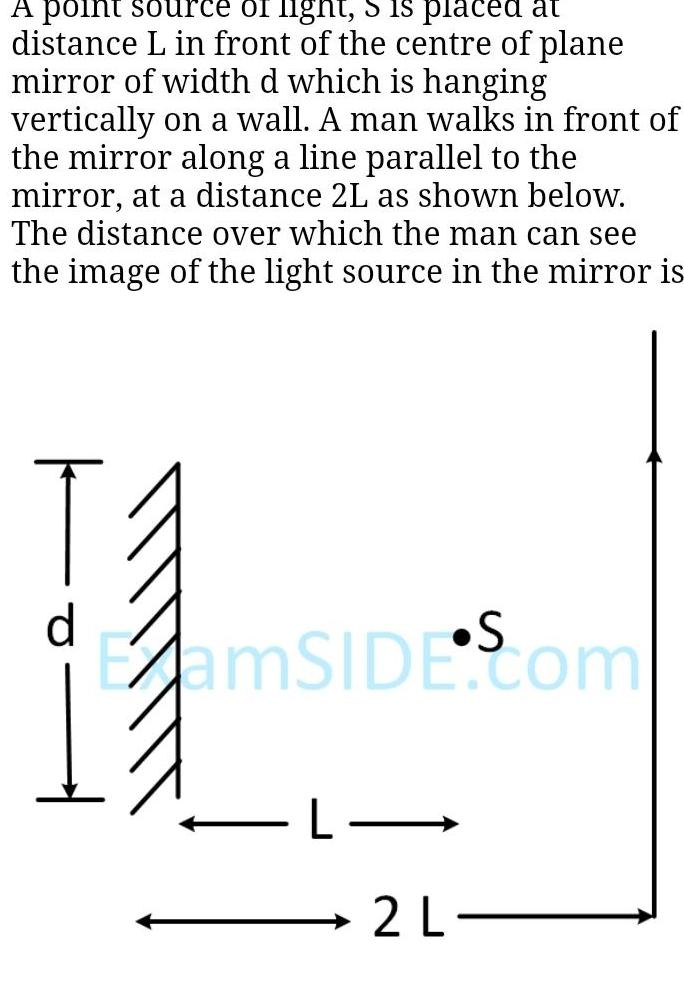Physics
Wave Optics
A point source of fight 5 is placed at distance L in front of the centre of plane mirror of width d which is hanging vertically on a wall A man walks in front of the mirror along a line parallel to the mirror at a distance 2L as shown below The distance over which the man can see the image of the light source in the mirror is d ExamSIDE Com L 2 L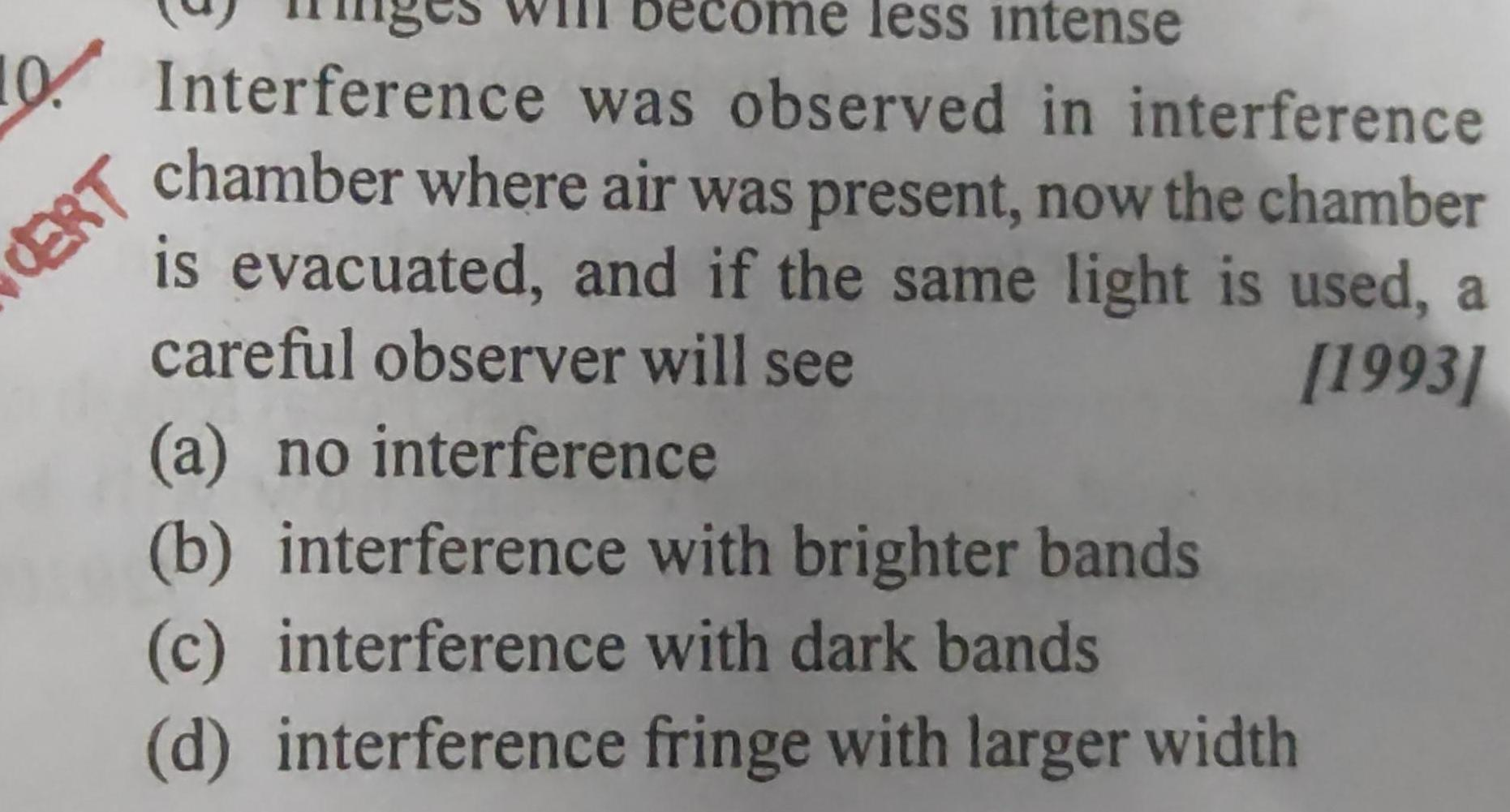Physics
Wave Optics
less intense 10 Interference was observed in interference chamber where air was present now the chamber is evacuated and if the same light is used a careful observer will see 1993 CERT a no interference b interference with brighter bands c interference with dark bands d interference fringe with larger width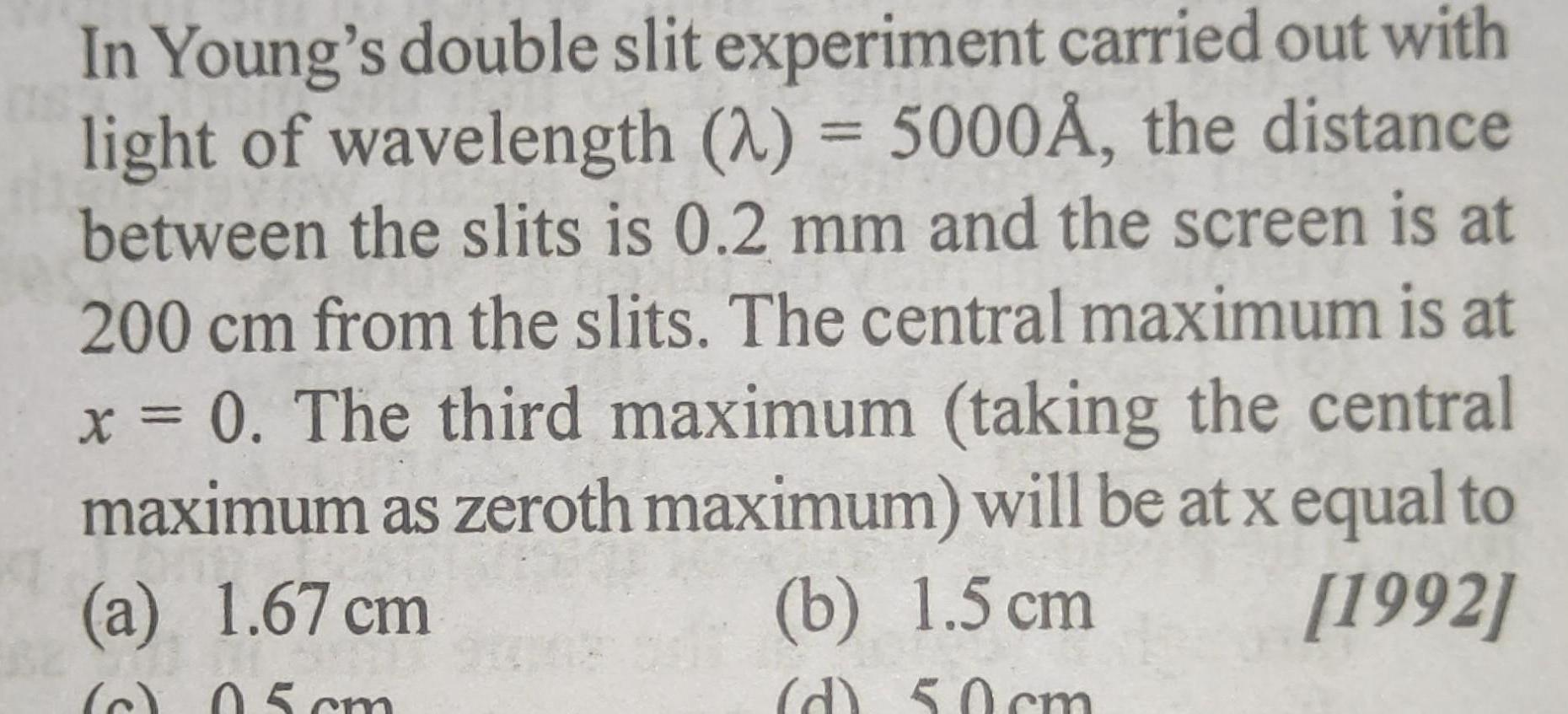Physics
Wave Optics
In Young s double slit experiment carried out with light of wavelength 2 5000 the distance between the slits is 0 2 mm and the screen is at 200 cm from the slits The central maximum is at x 0 The third maximum taking the central maximum as zeroth maximum will be at x equal to a 1 67 cm b 1 5 cm 1992 c 05cm d 50 cm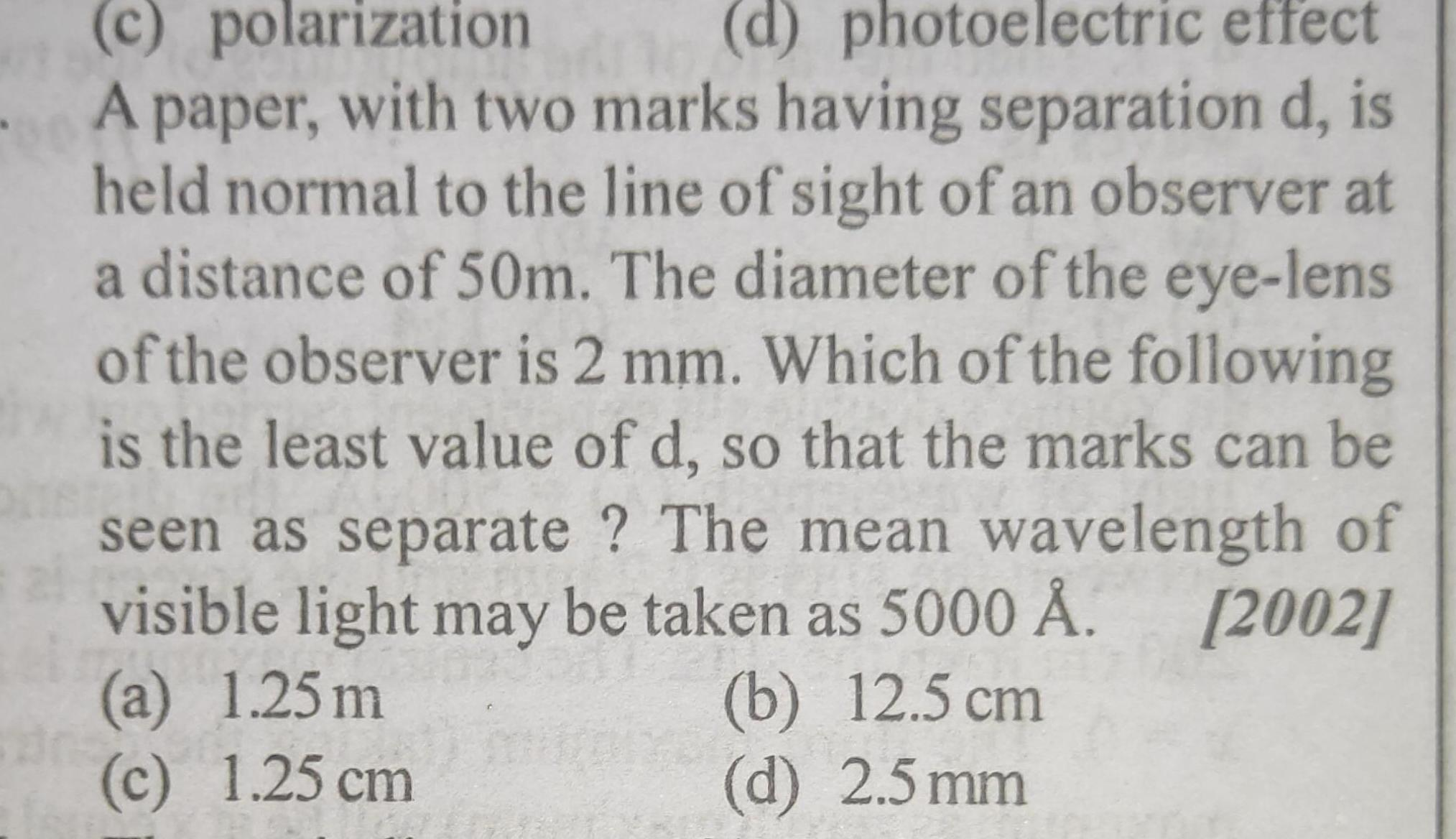Physics
Wave Optics
c polarization d photoelectric effect A paper with two marks having separation d is held normal to the line of sight of an observer at a distance of 50m The diameter of the eye lens of the observer is 2 mm Which of the following is the least value of d so that the marks can be seen as separate The mean wavelength of visible light may be taken as 5000 2002 a 1 25m c 1 25 cm b 12 5 cm d 2 5 mm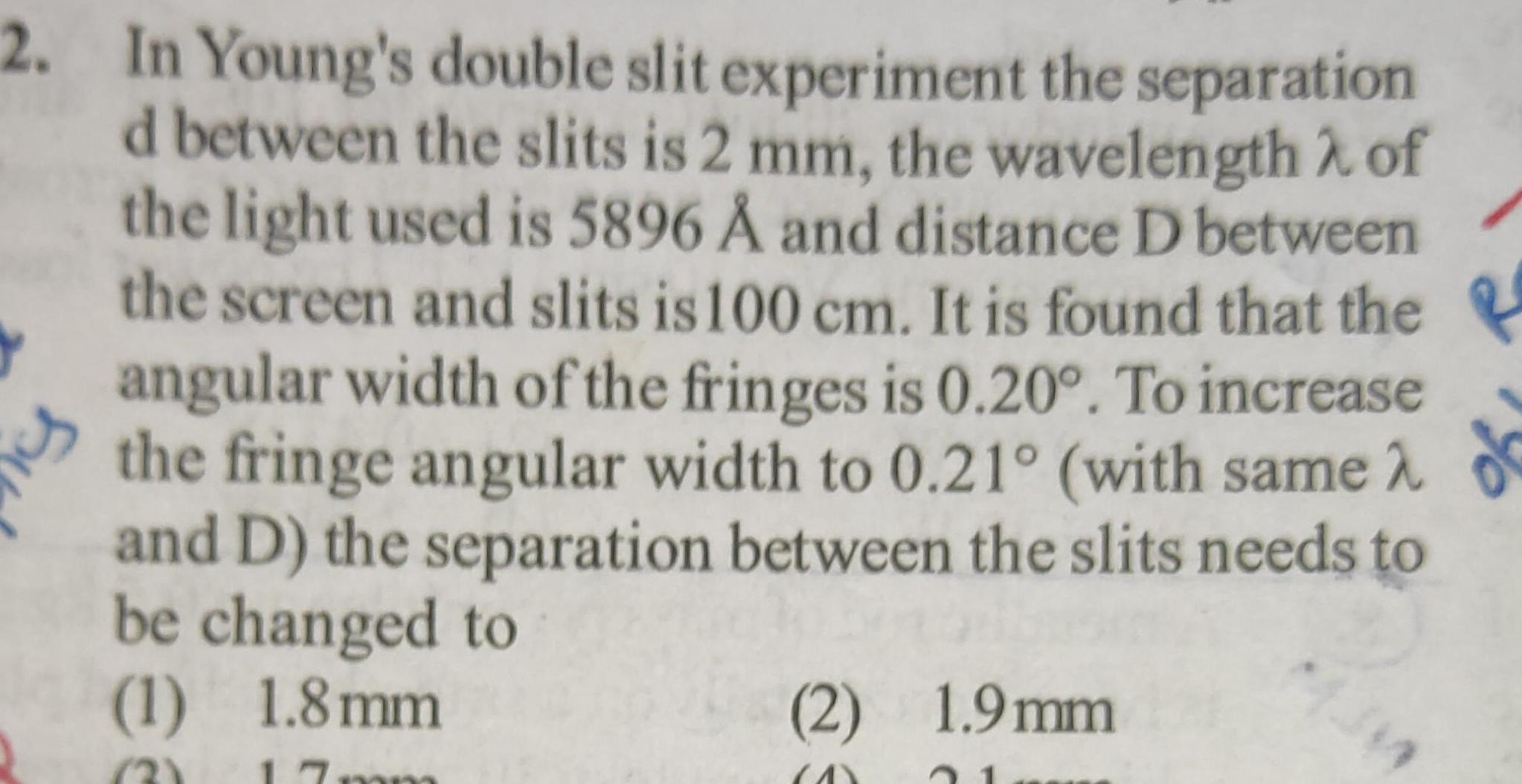Physics
Wave Optics
2 In Young s double slit experiment the separation d between the slits is 2 mm the wavelength of the light used is 5896 and distance D between the screen and slits is 100 cm It is found that the angular width of the fringes is 0 20 To increase the fringe angular width to 0 21 with same and D the separation between the slits needs to be changed to 1 1 8mm R 2 17mm f 2 1 9mm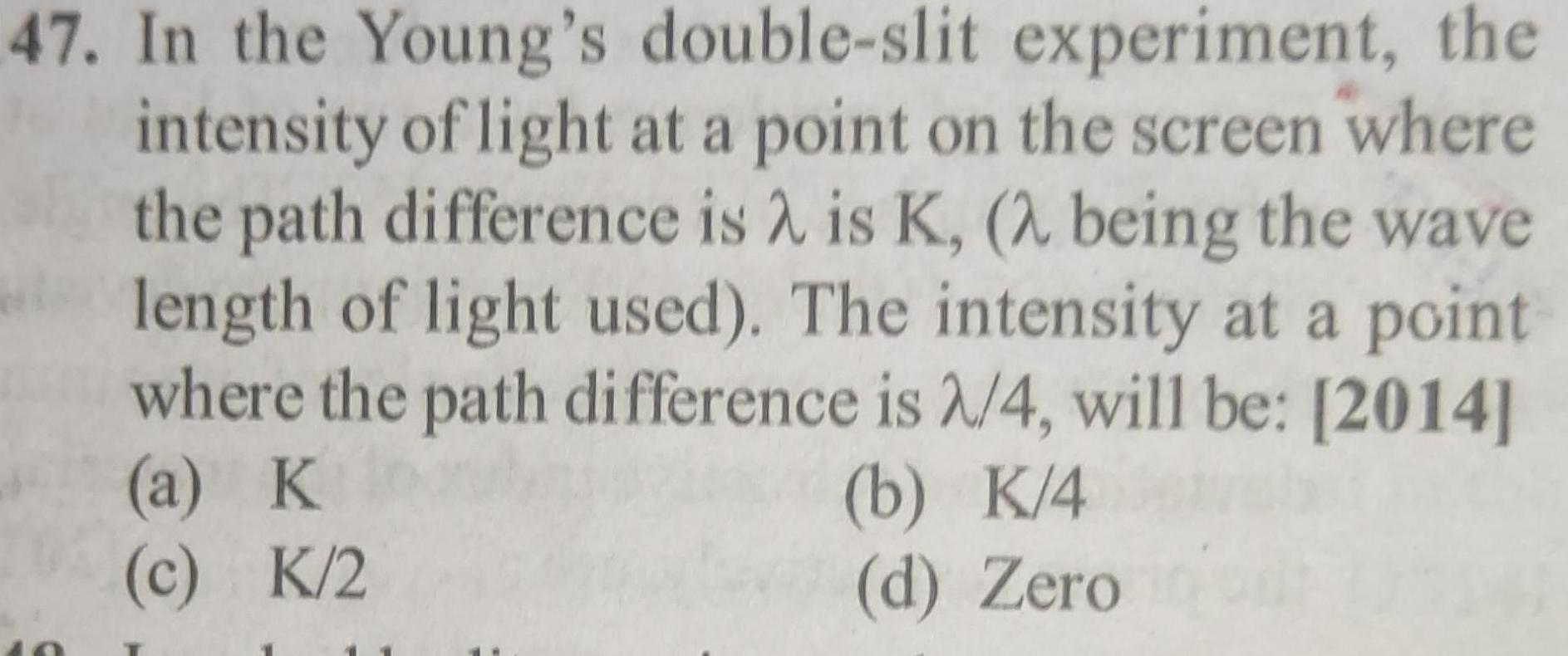Physics
Wave Optics
47 In the Young s double slit experiment the intensity of light at a point on the screen where the path difference is is K 2 being the wave length of light used The intensity at a point where the path difference is 2 4 will be 2014 a K c K 2 b K 4 d Zero